## Sunday, December 30, 2018

2014
(November)
MATHEMATICS
(Major)
Course: 502
(Linear Algebra and Number Theory)
Full Marks: 80
Pass Marks: 32
Time: 3 hours
The figures in the margin indicate full marks for the questions
GROUP – A
(Linear Algebra)
(Marks: 40)

1. Answer the following questions: 1x4=4
1. Define subspace of a vector space.
2. Let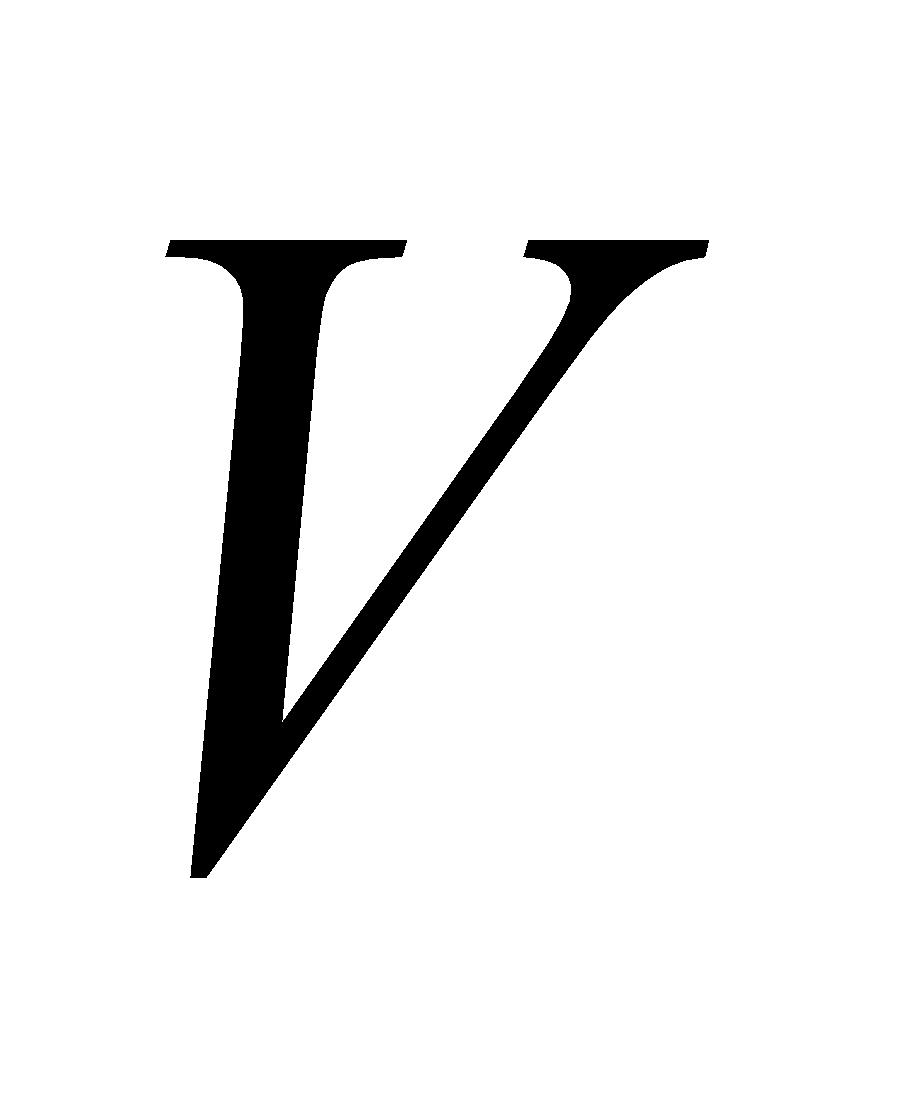be a vector space over same field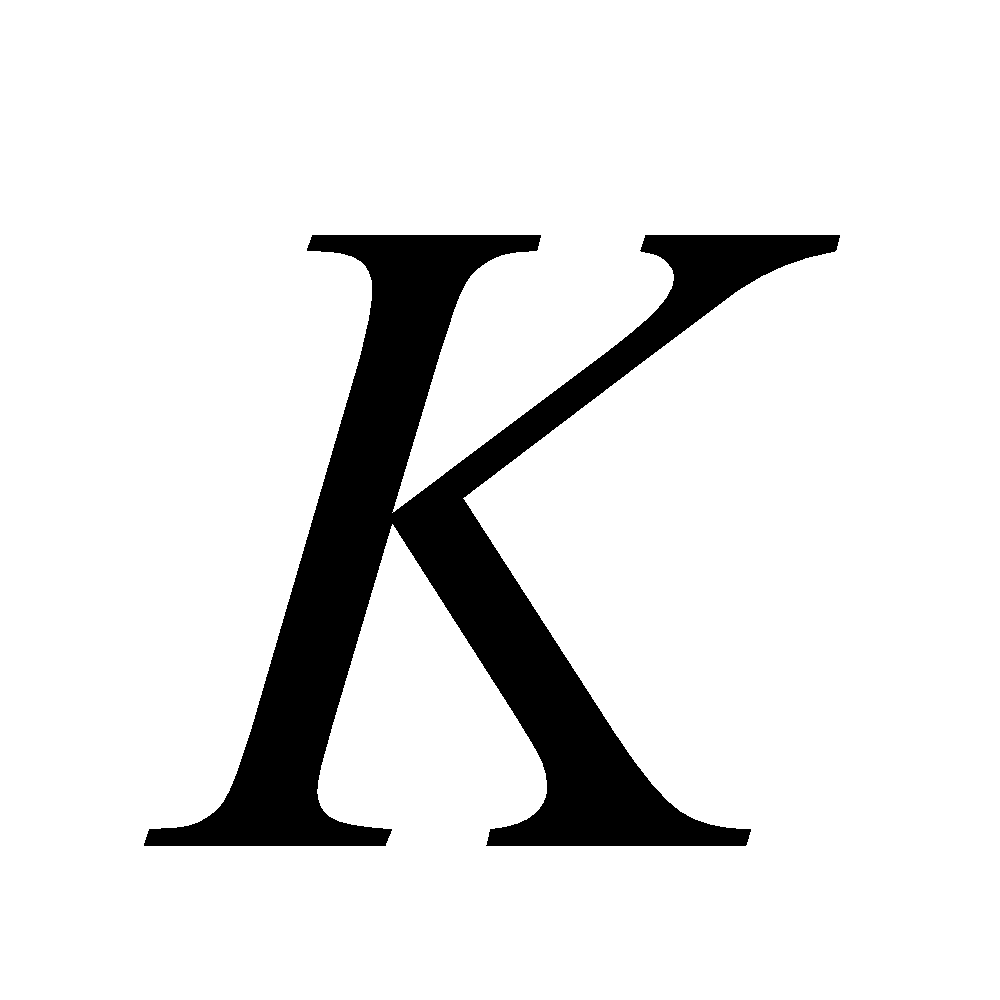. Show that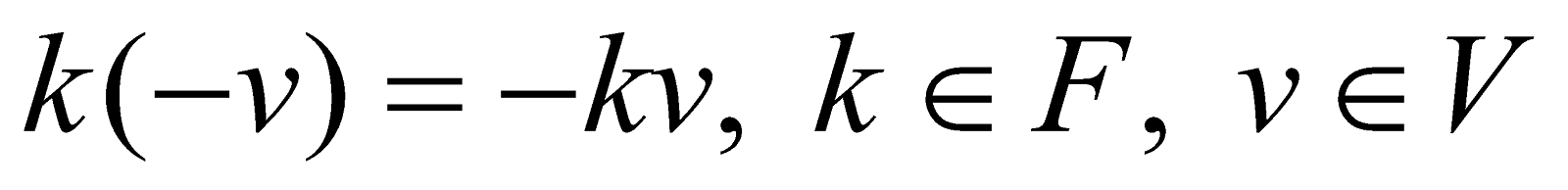1. Define kernel of a linear mapping.
2. Find the matrix of the linear operator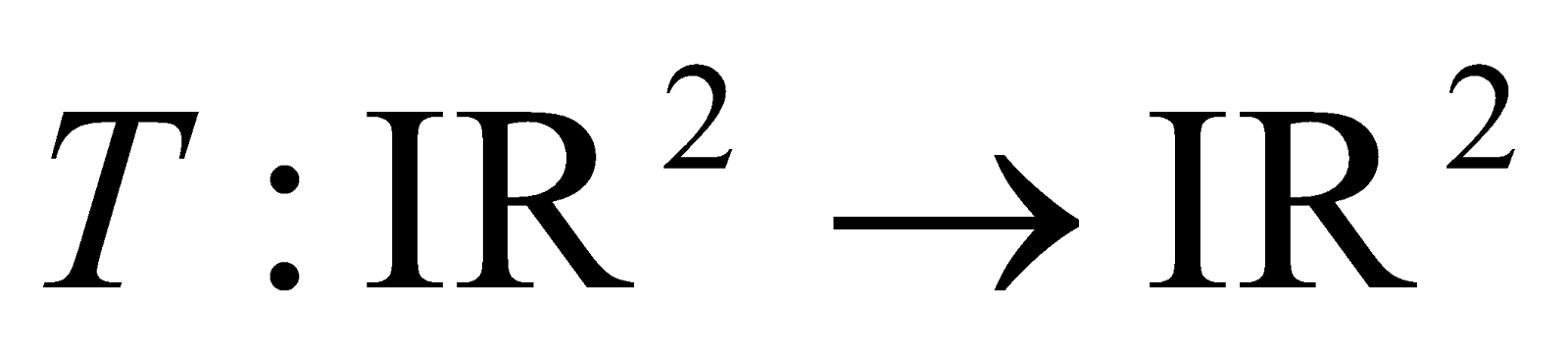defined by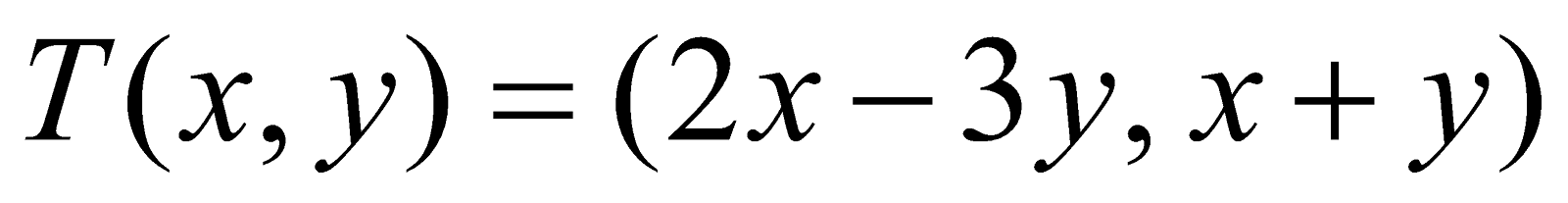with the usual basis.
1. Determine whether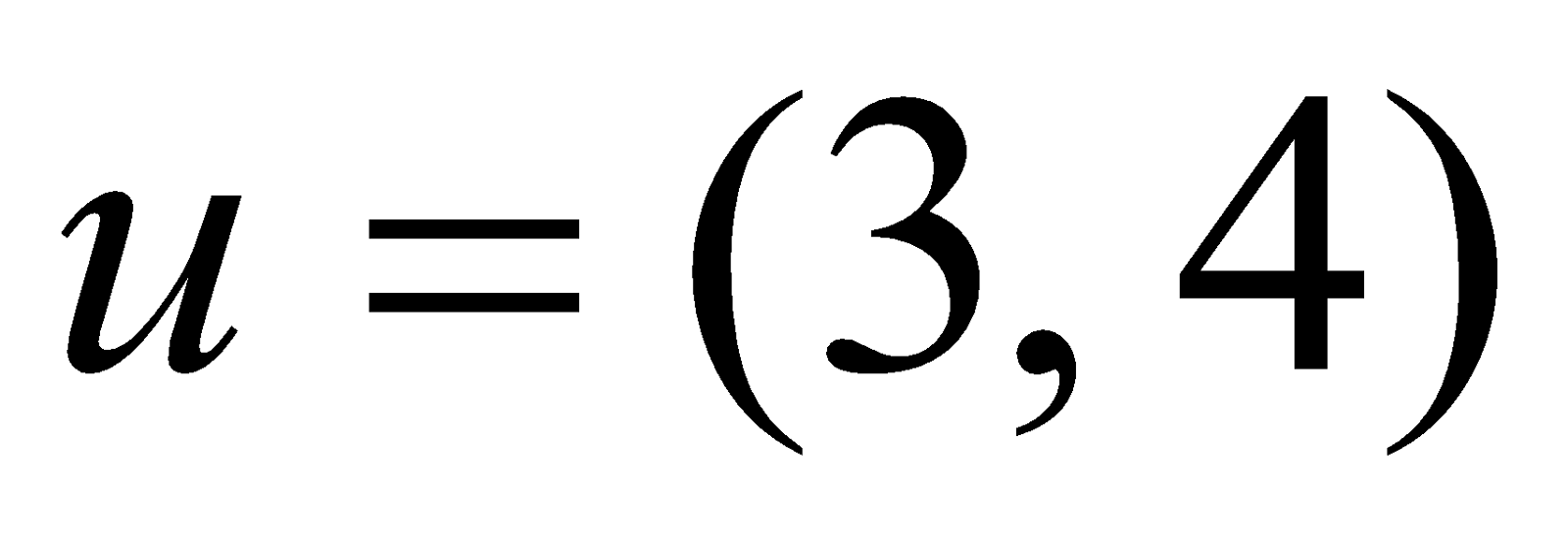and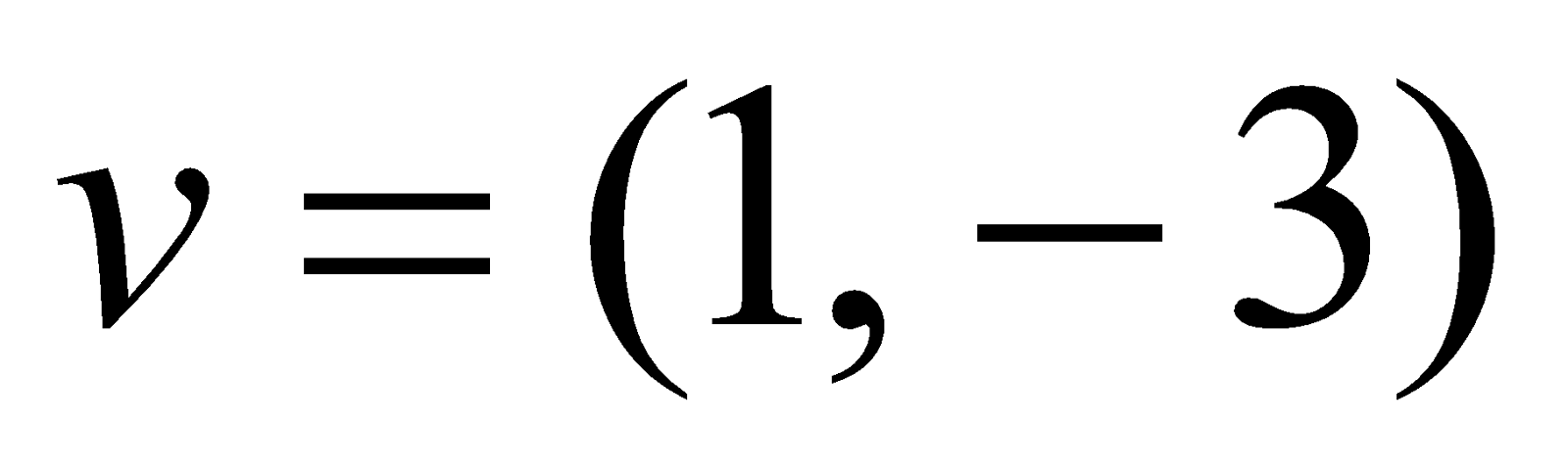are linearly dependent. 2
2. Letbe a vector space overand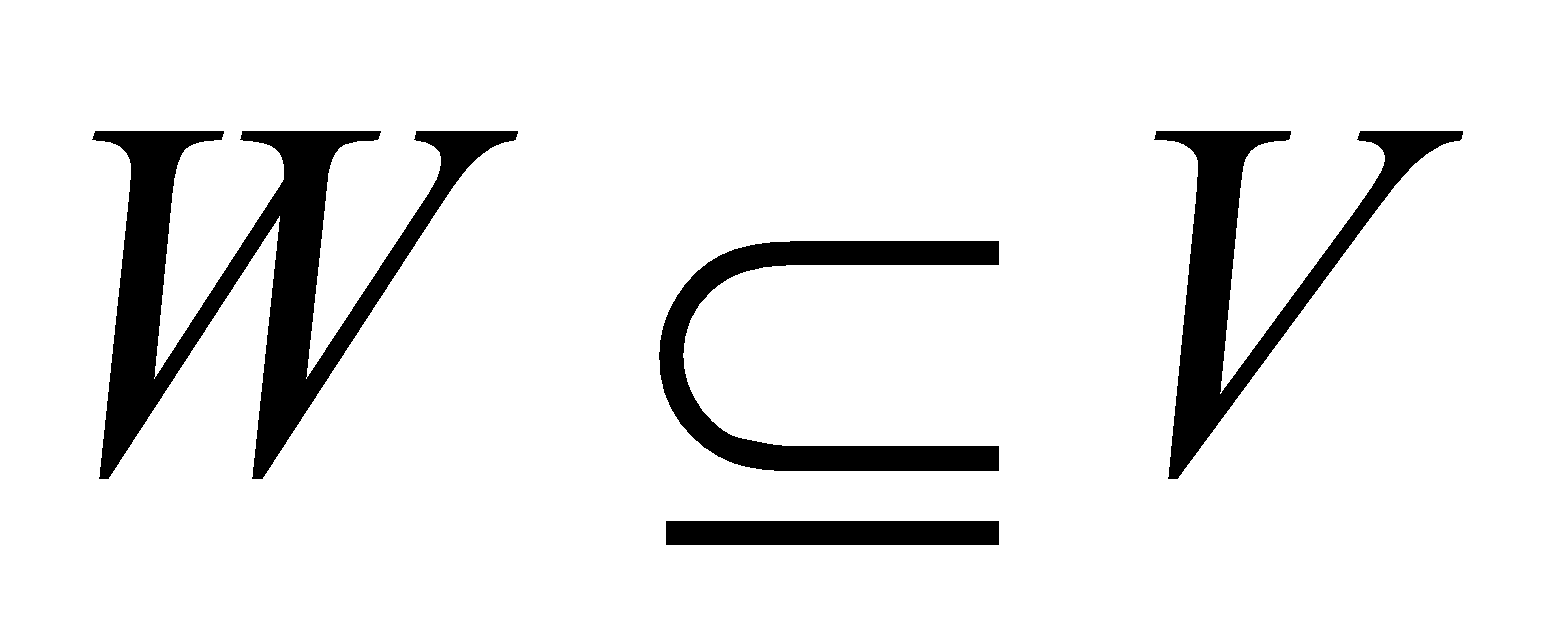. Show that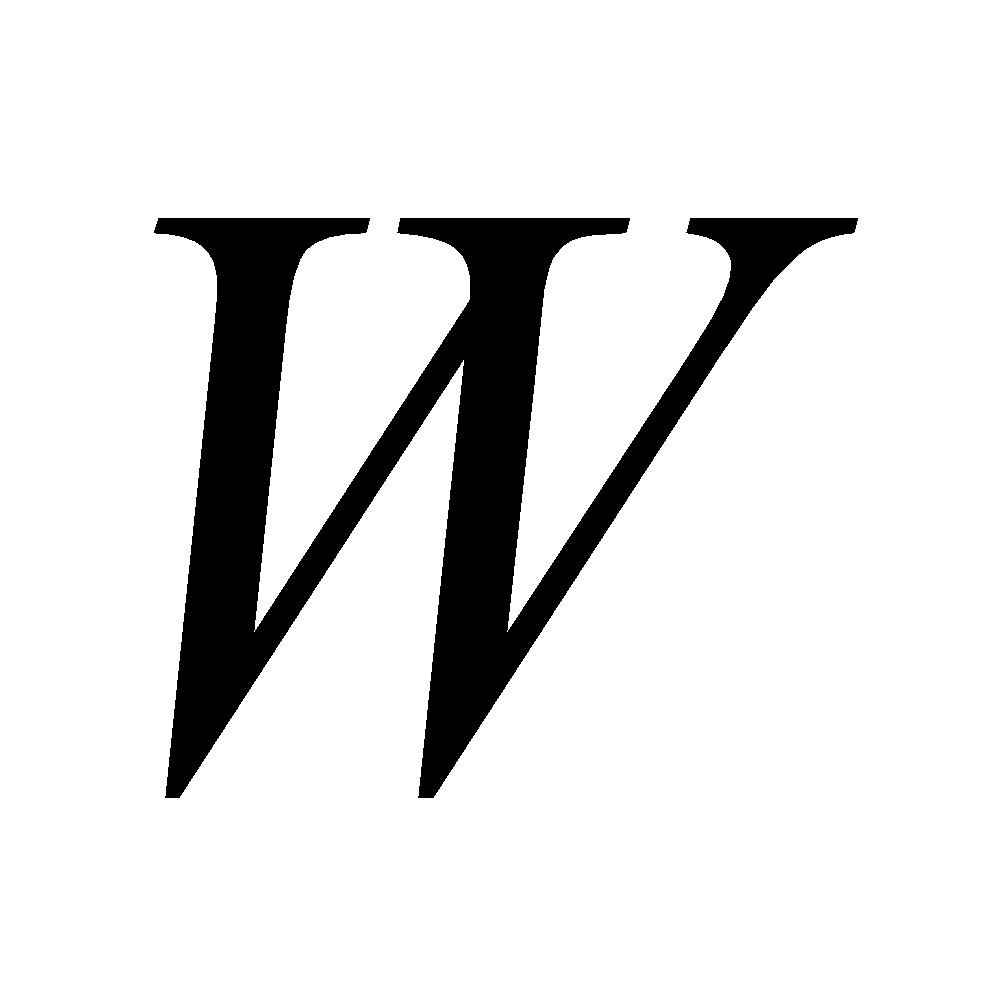is a subspace ofiff
1.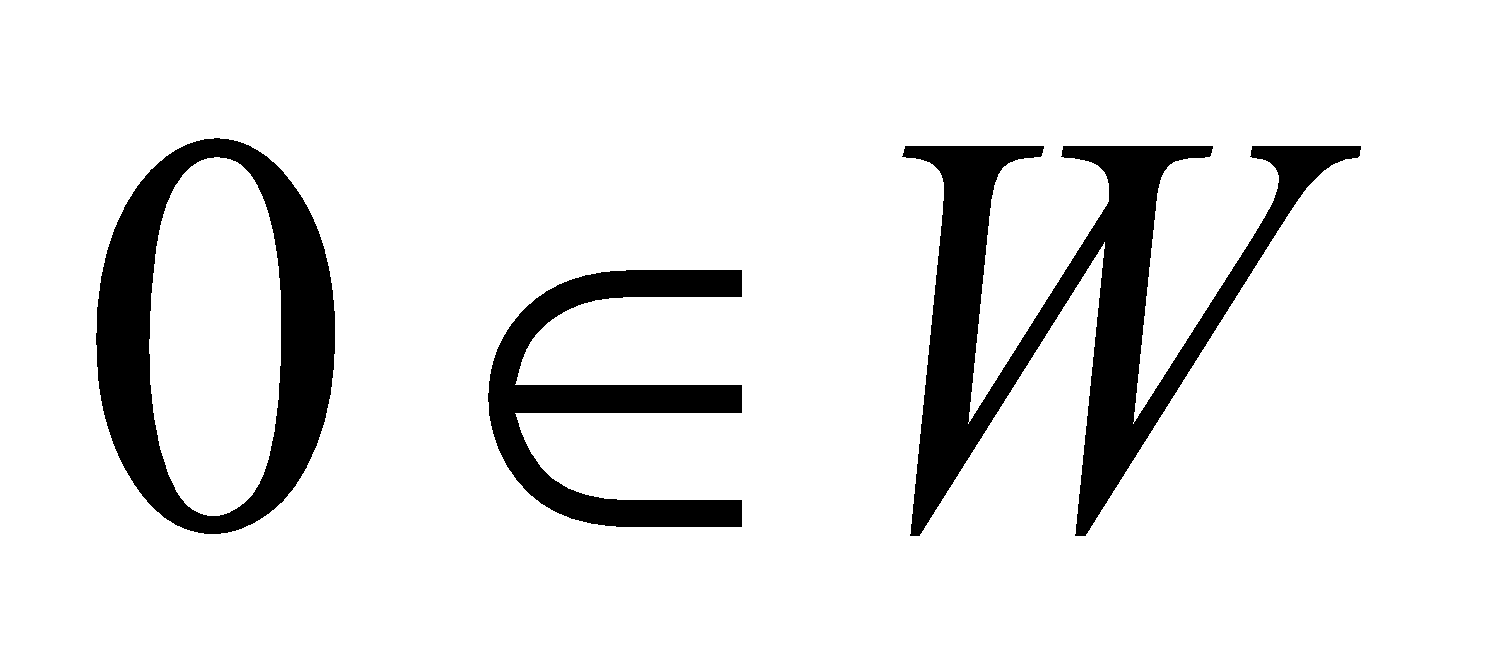;
2.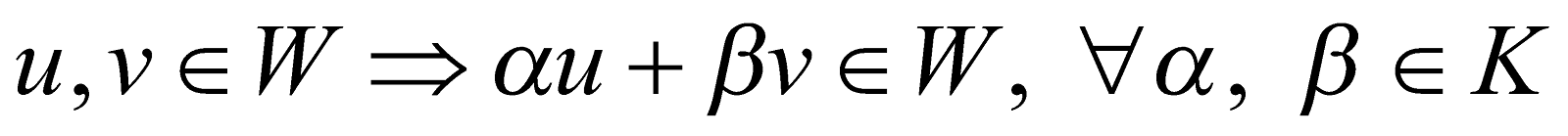. 3
1. Find, for what value of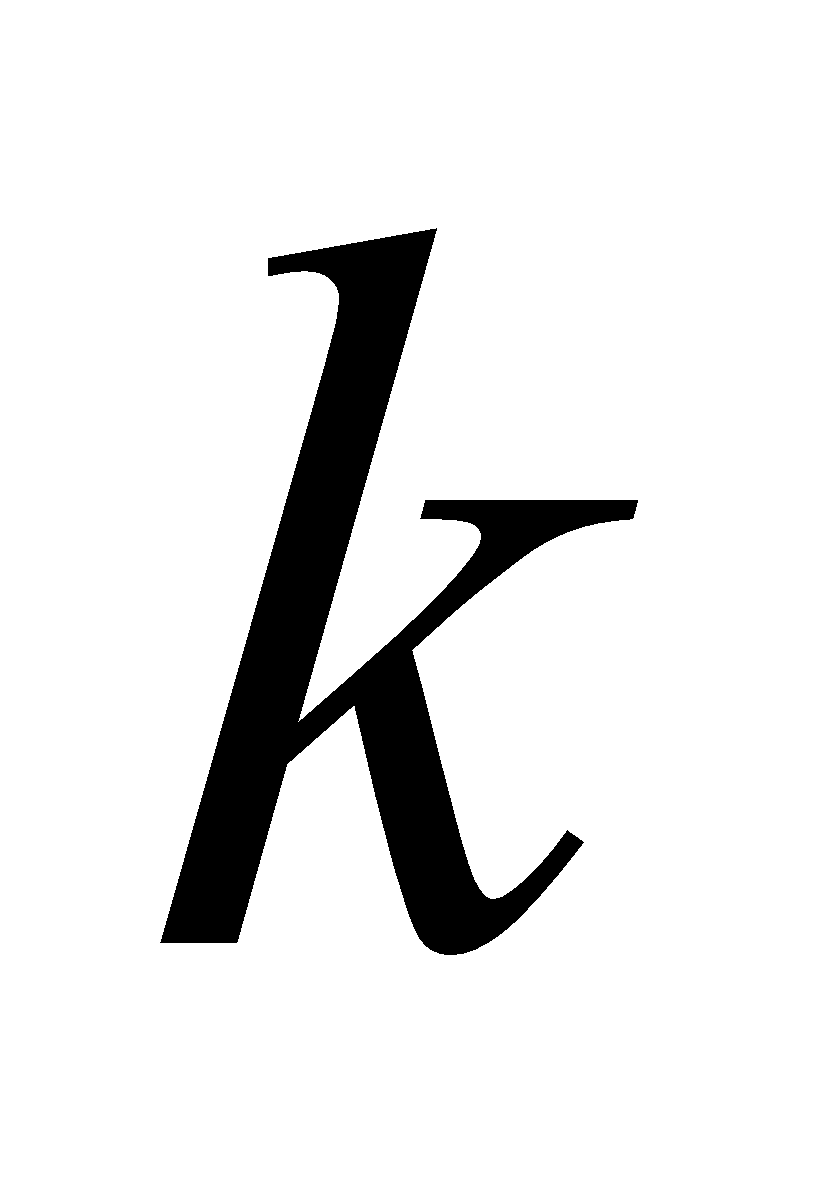, the vector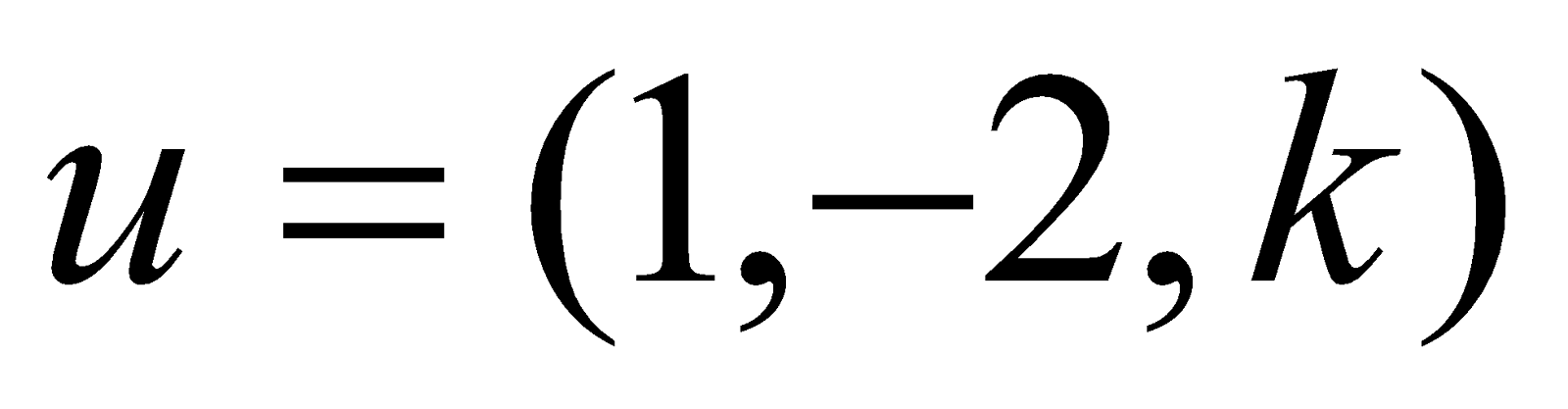in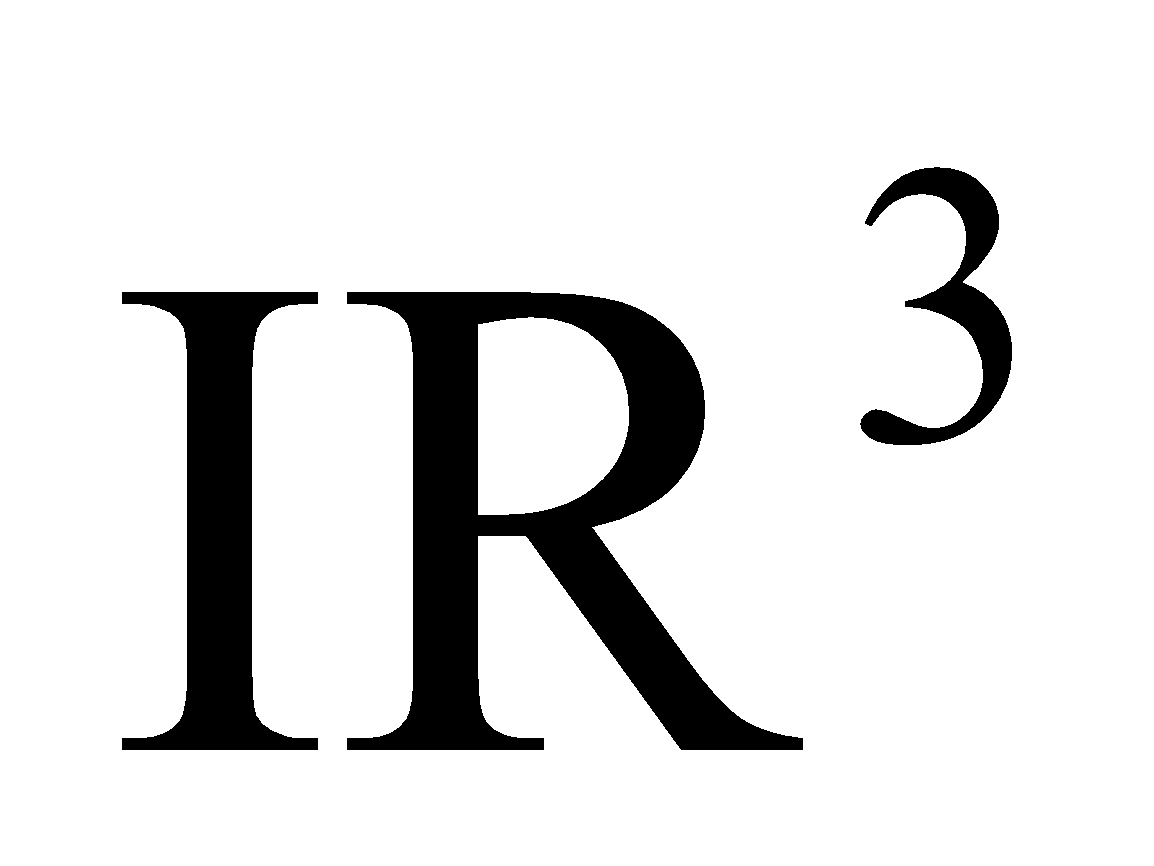is a linear combination of the vectors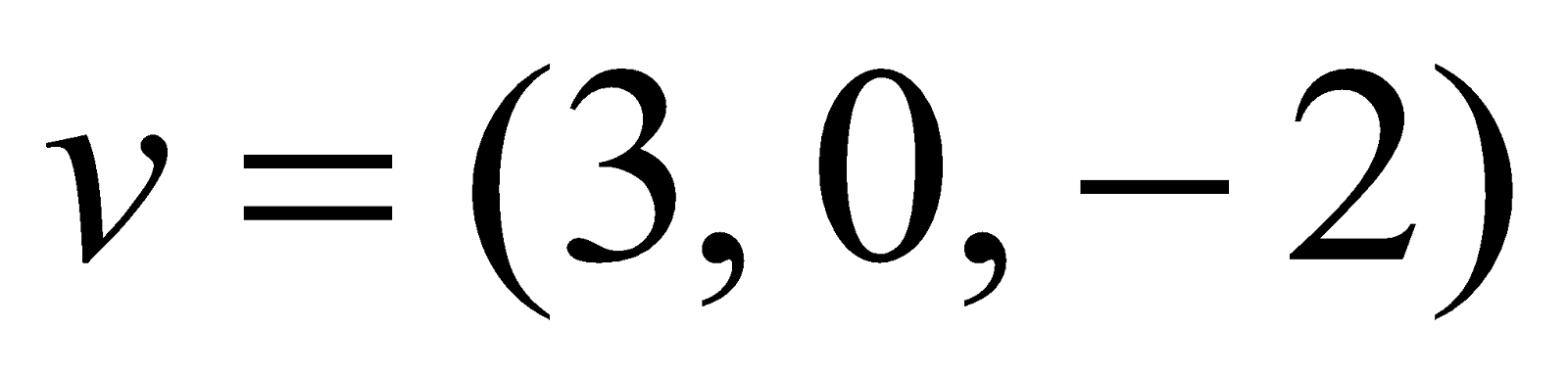and. 3
2. Letbe the subspace of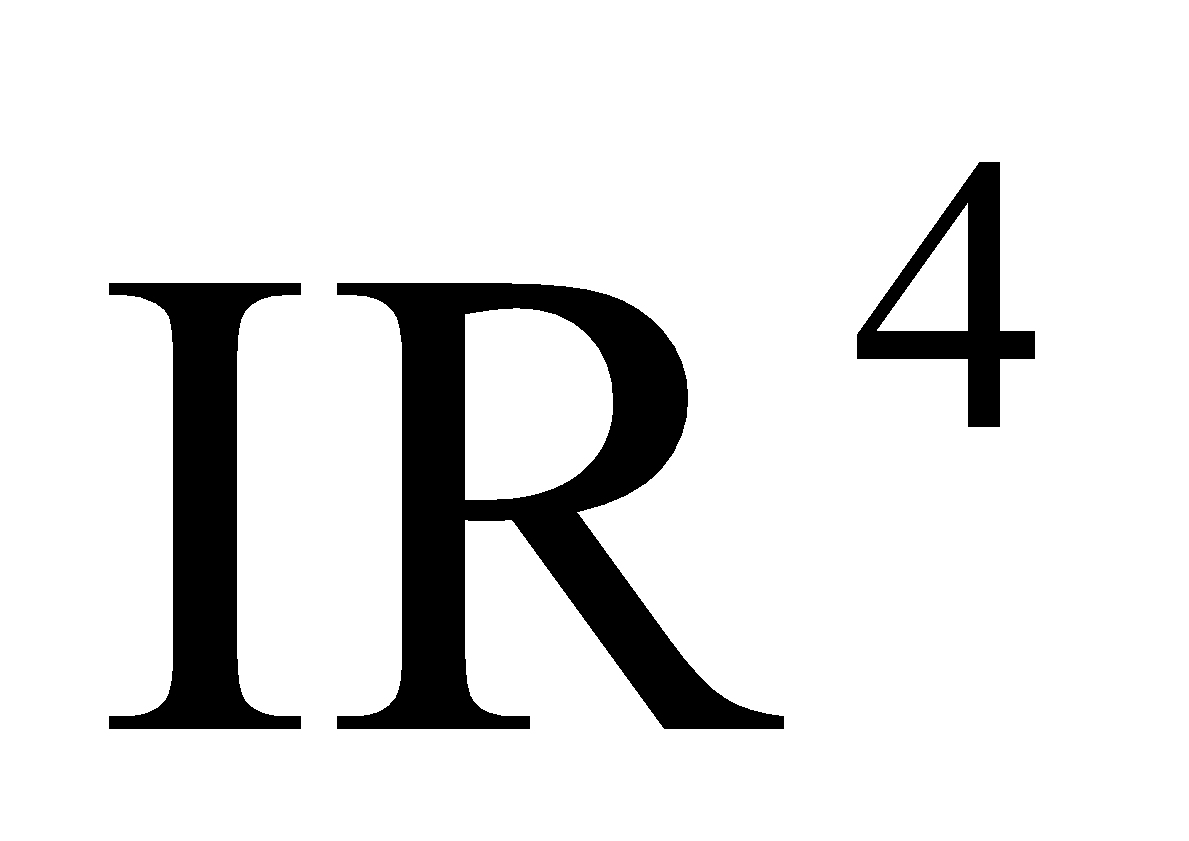generated by the vectors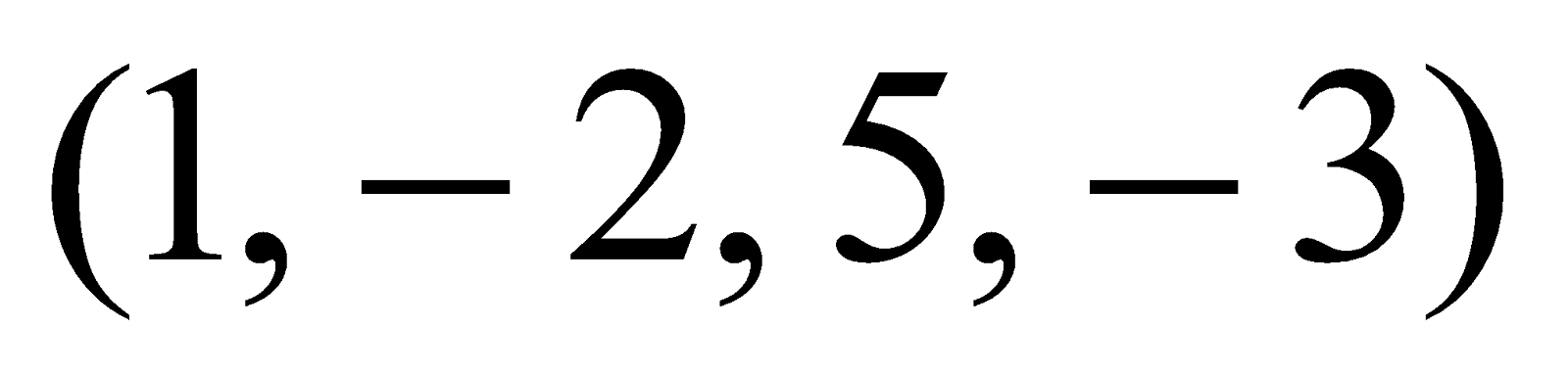,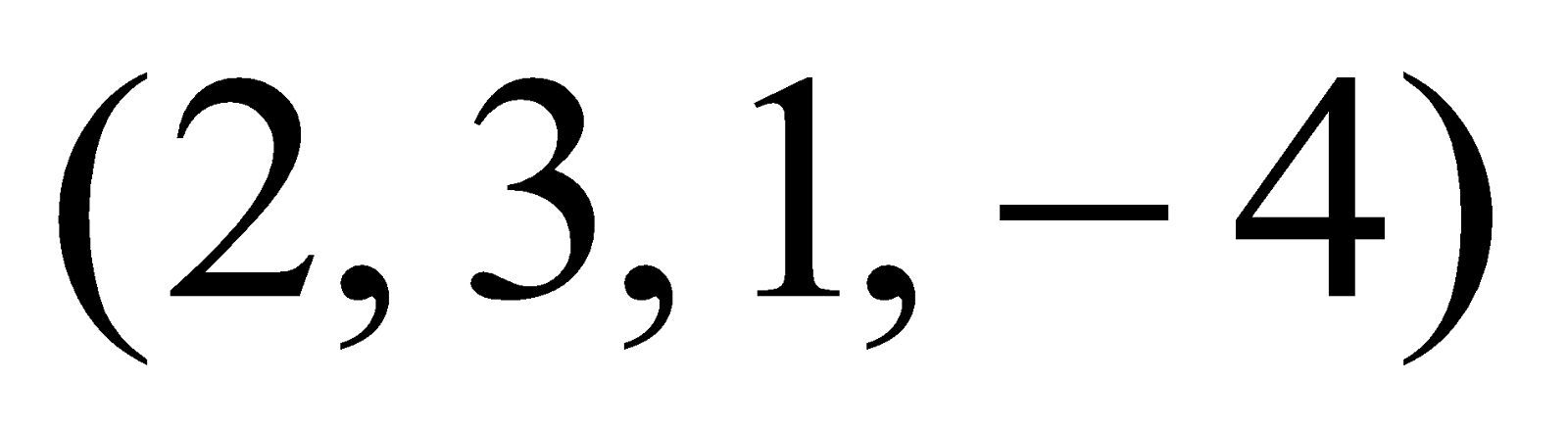,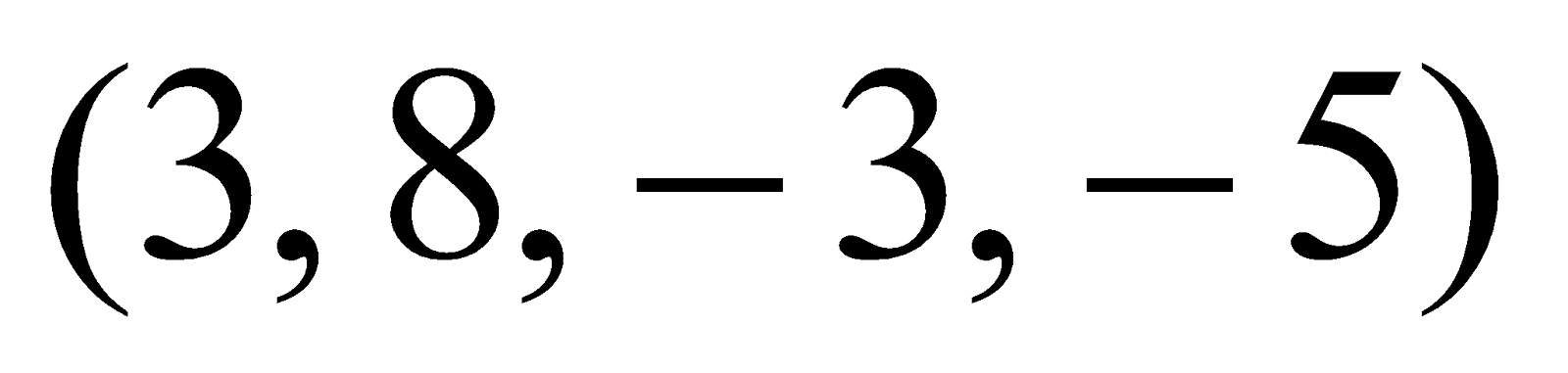. Find a basis of.
3. Prove that a vector space ofis the direct sum of its subspaces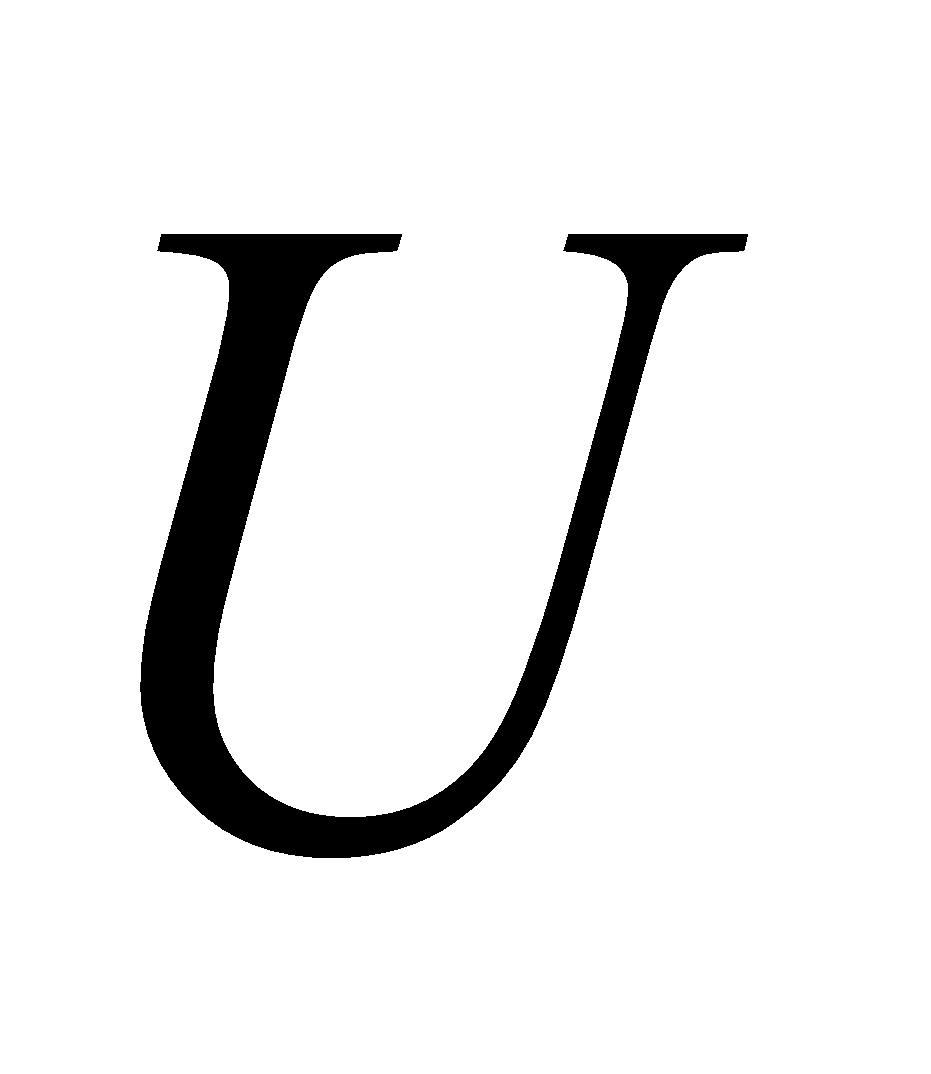and, iff
1.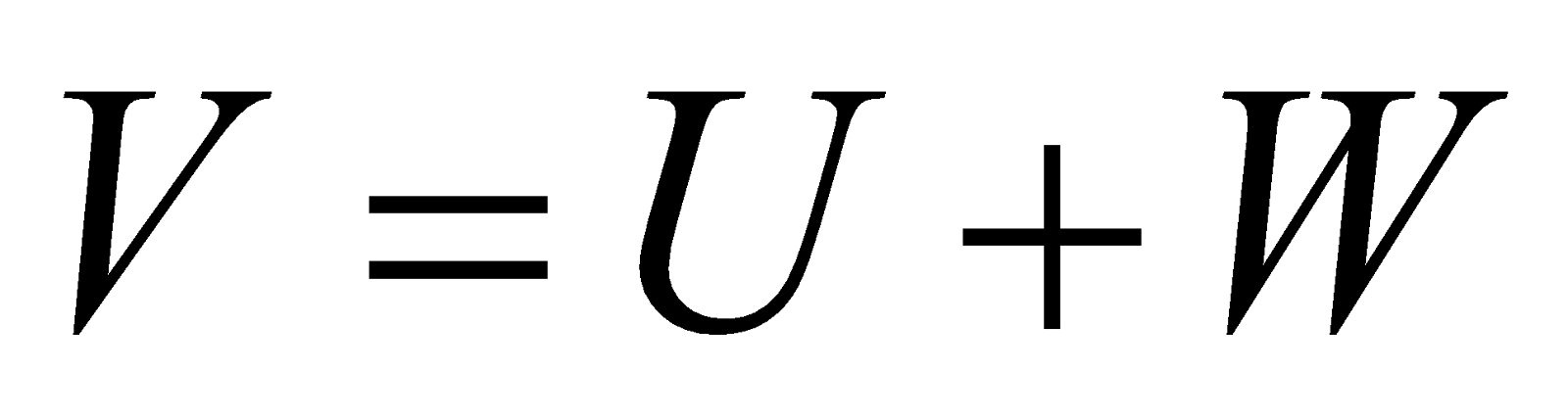2.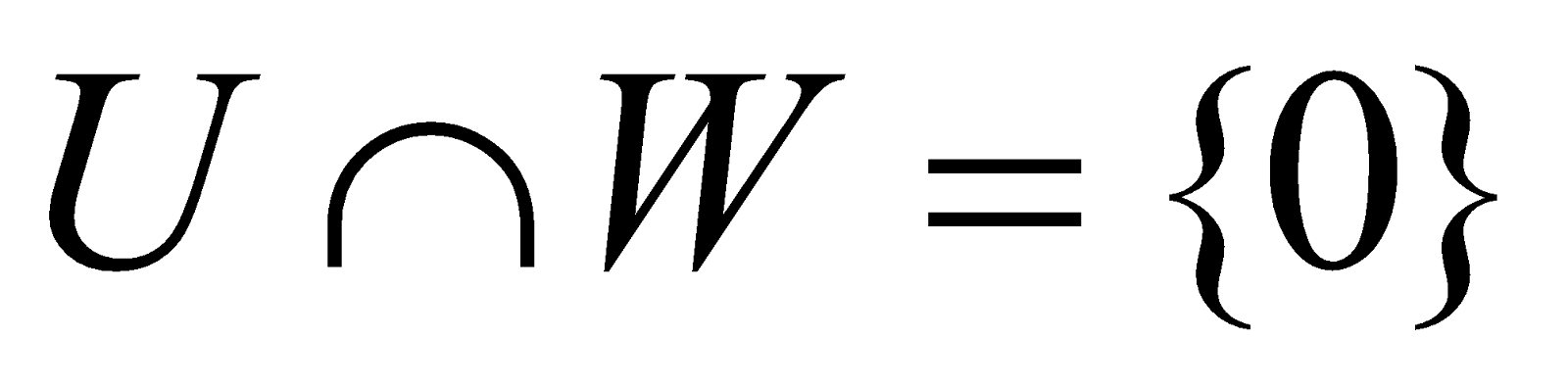1. Examine whether the following mapping are linear or not: 4
1.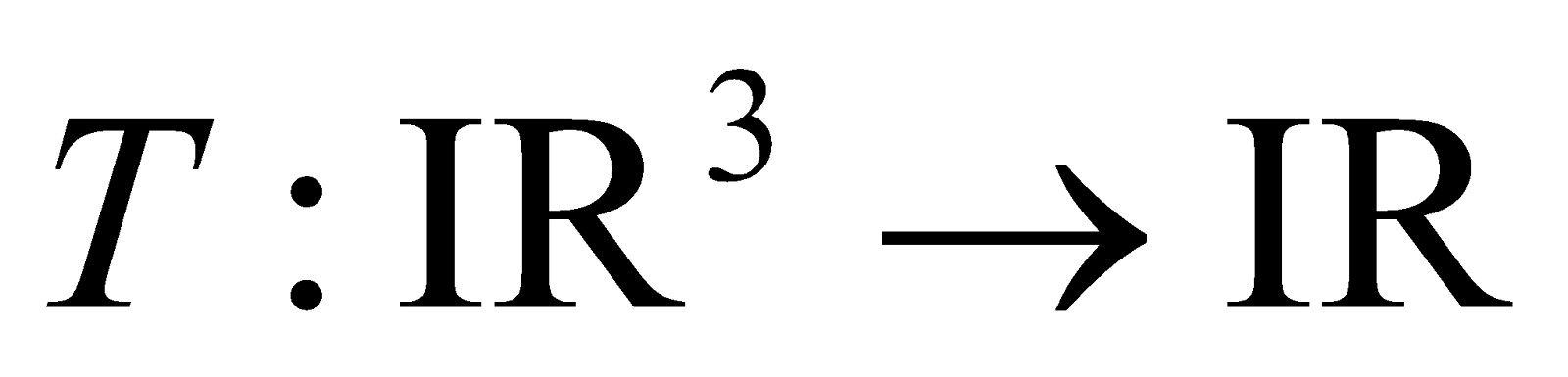defined by1.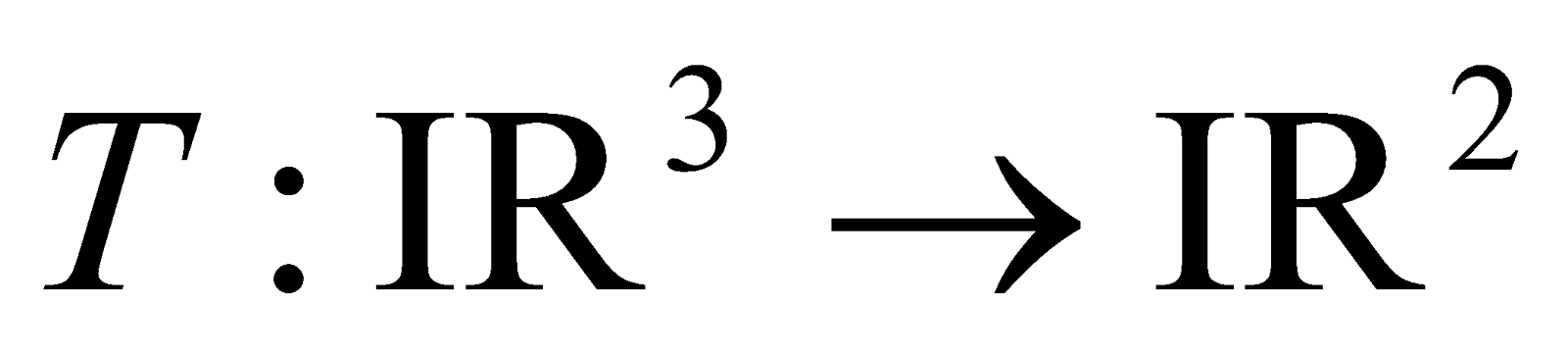defined by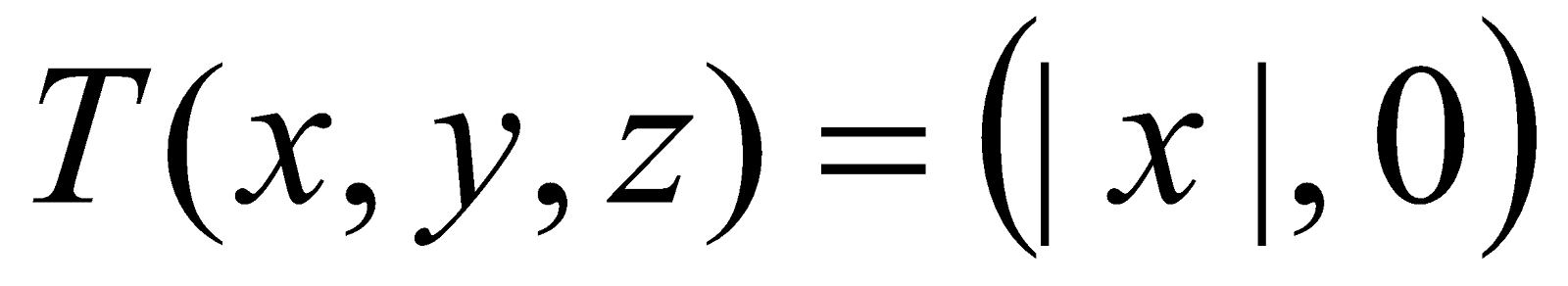3. Answer any three of the following questions: 6x3=18
1. Show that any two bases of a finite-dimensional vector space have same number of elements.
2. Let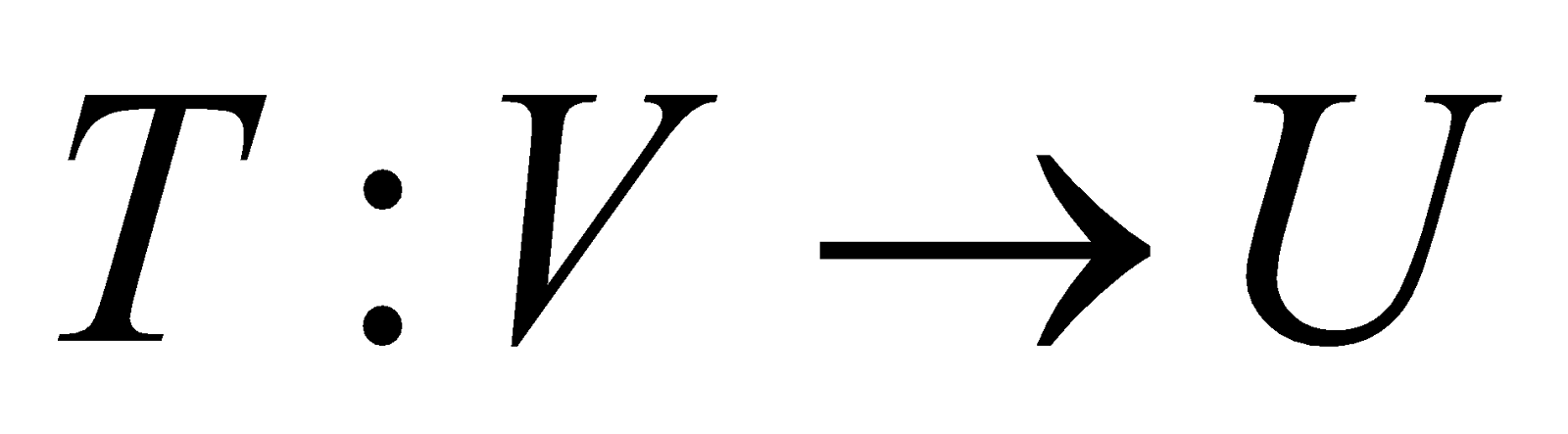be a linear transformation.
Show that
dim= rank of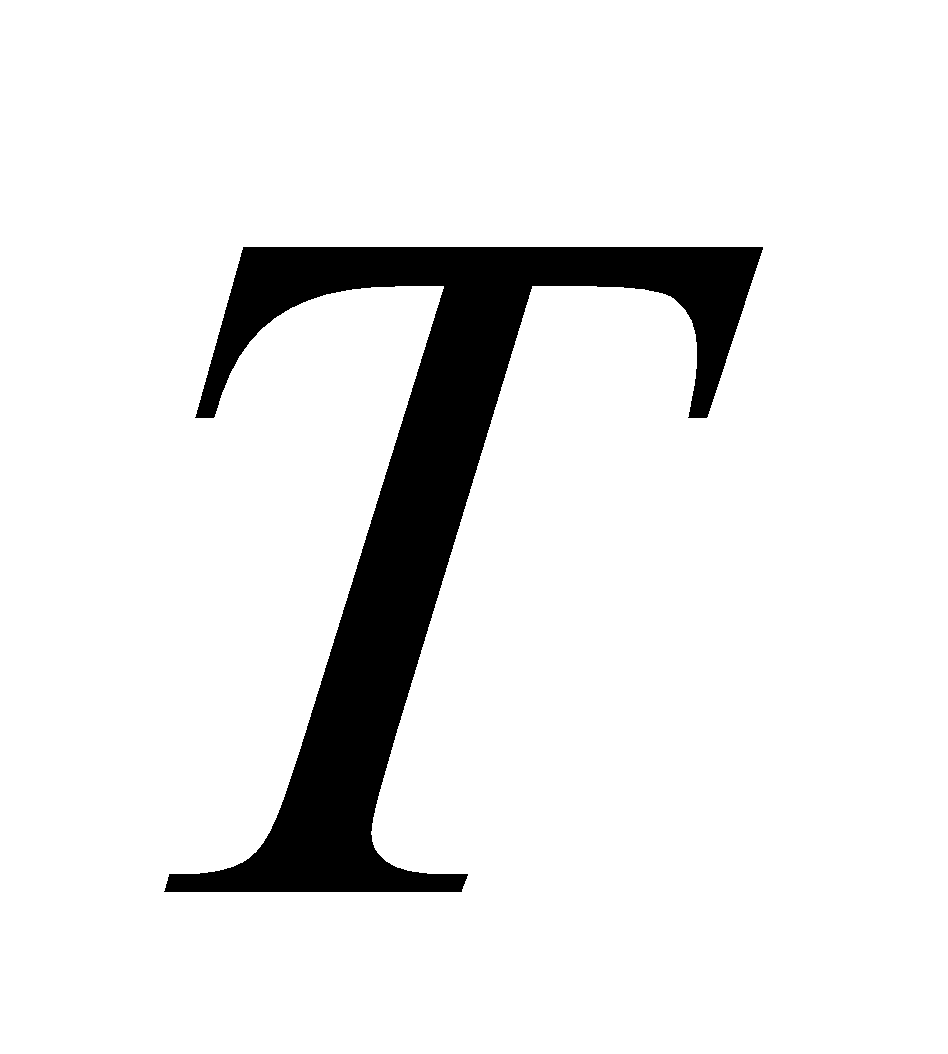+ nullity of1. Ifis a finite-dimensional vector space ofis a subspace of, then show that
dim= dim- dim.
1. Prove that the rows ranks and the column rank of an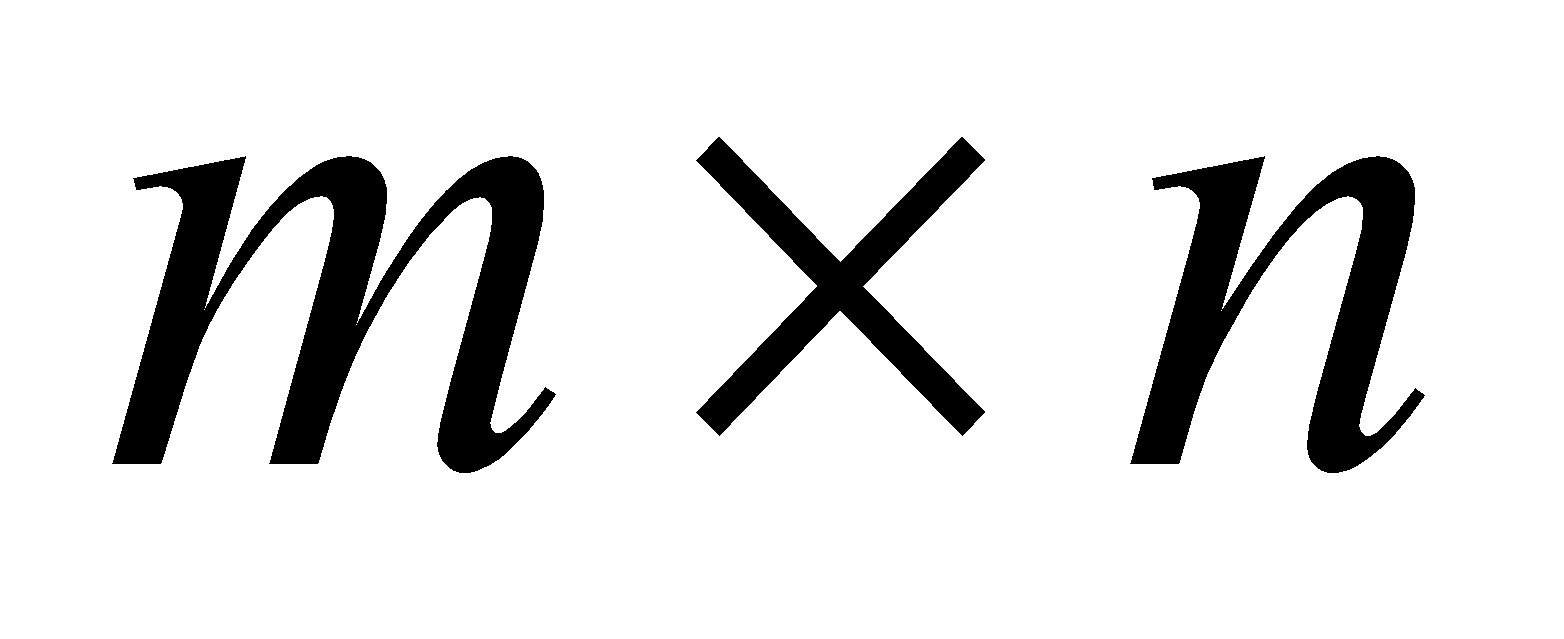matrix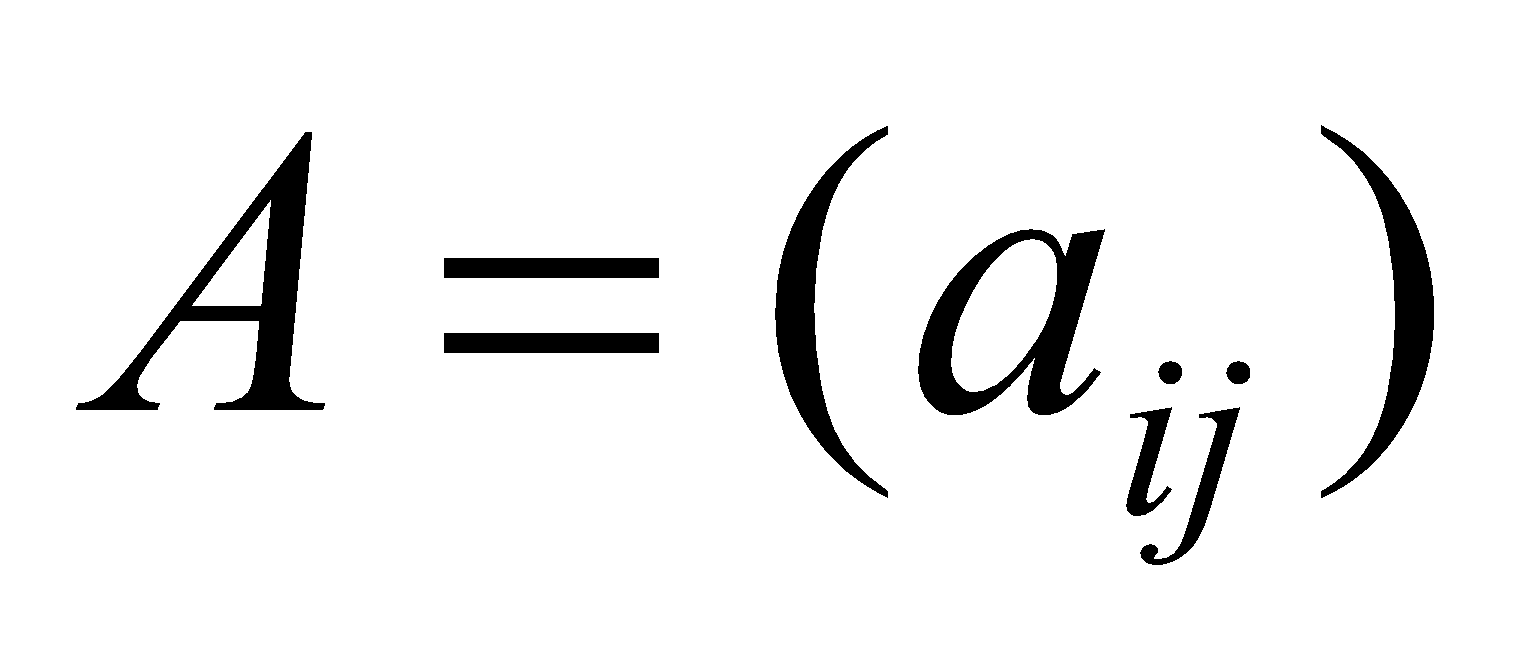are equal.

GROUP – B
(Number Theory)
(Marks: 40)
4. Answer the following questions: 1x4=4
1. If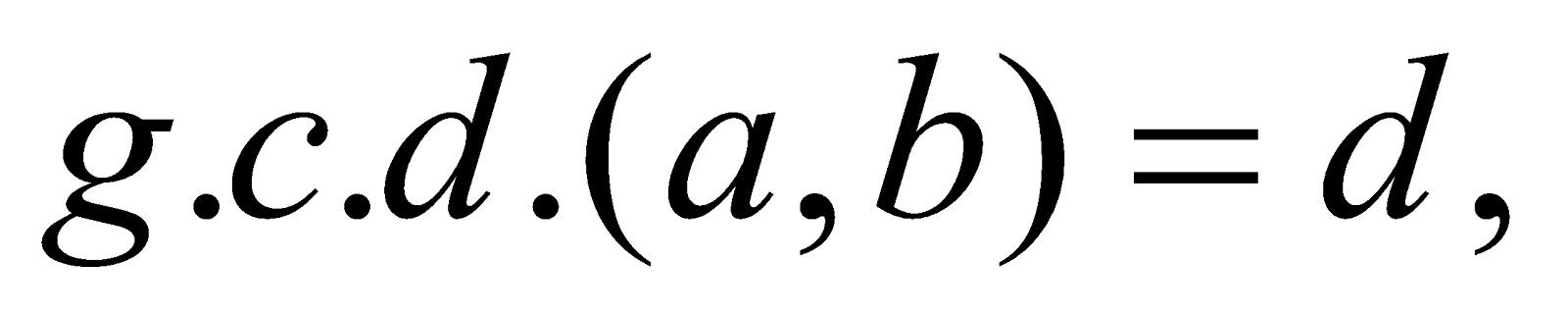then show that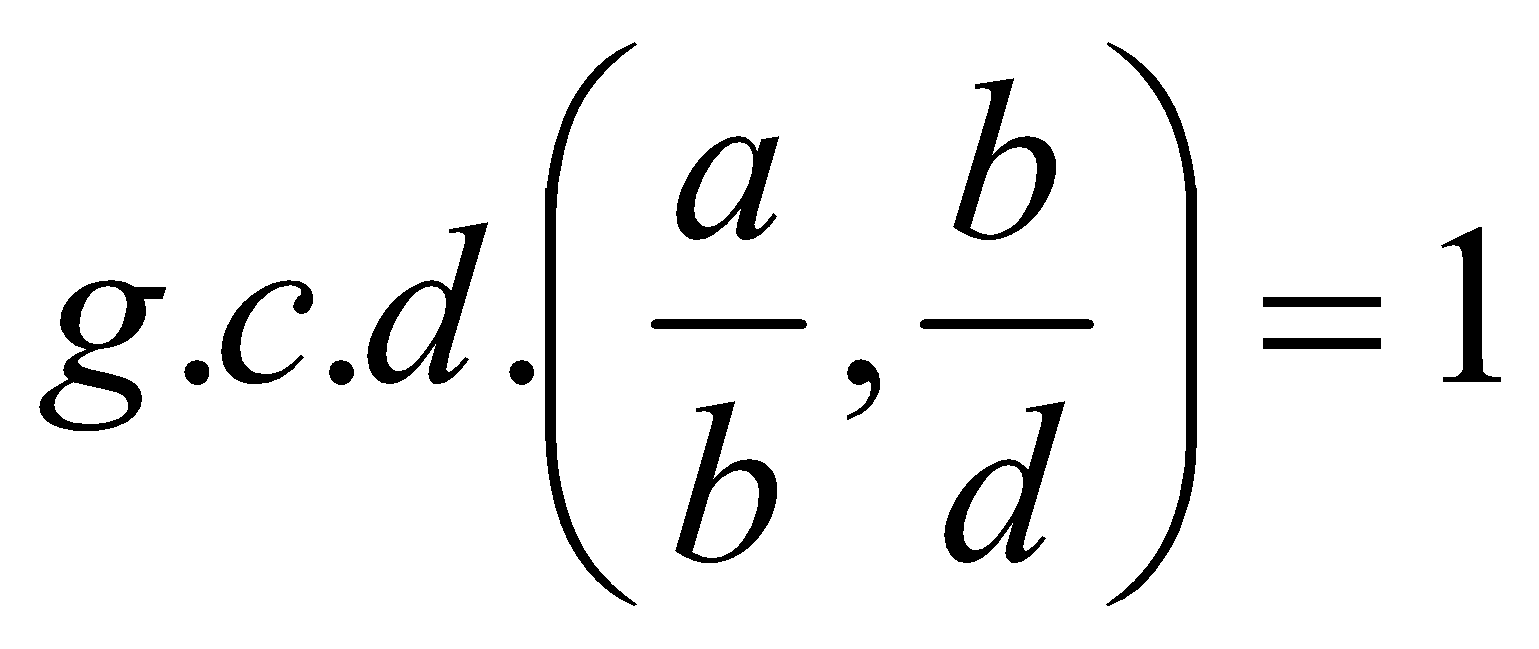1. If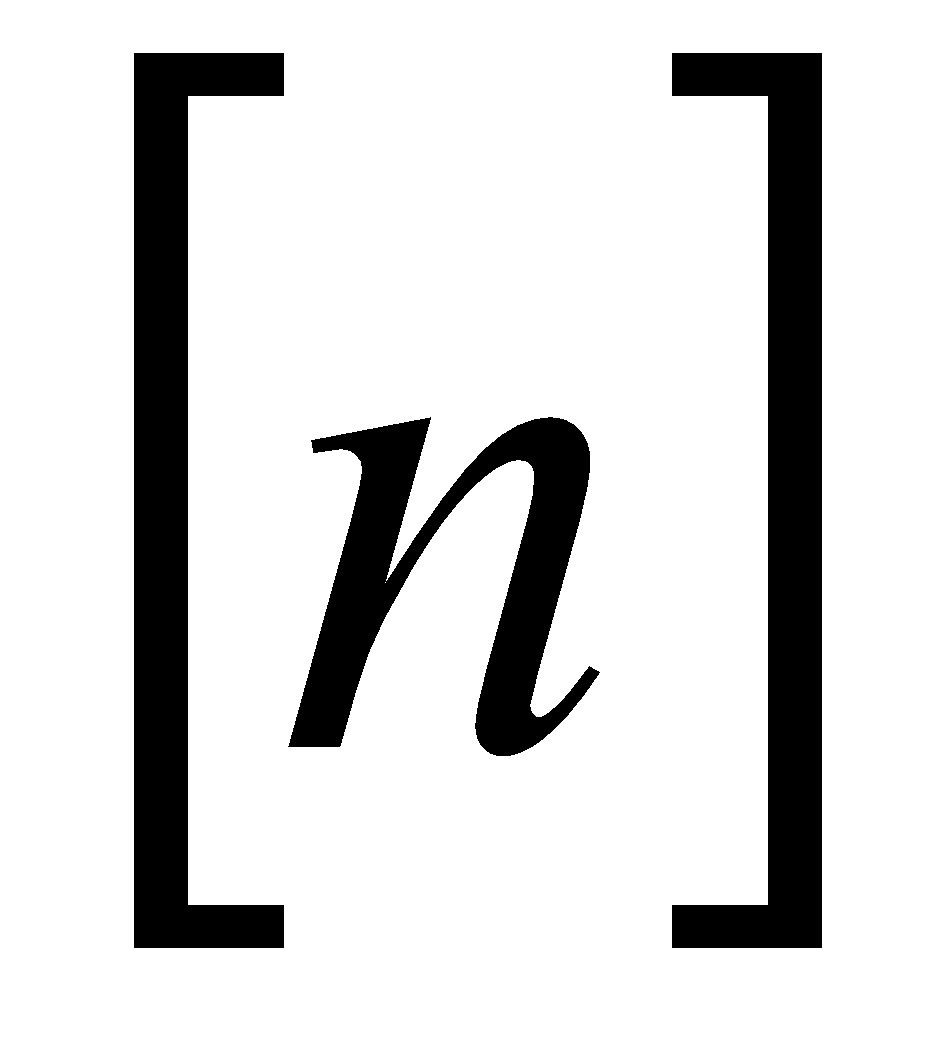denotes the largest integer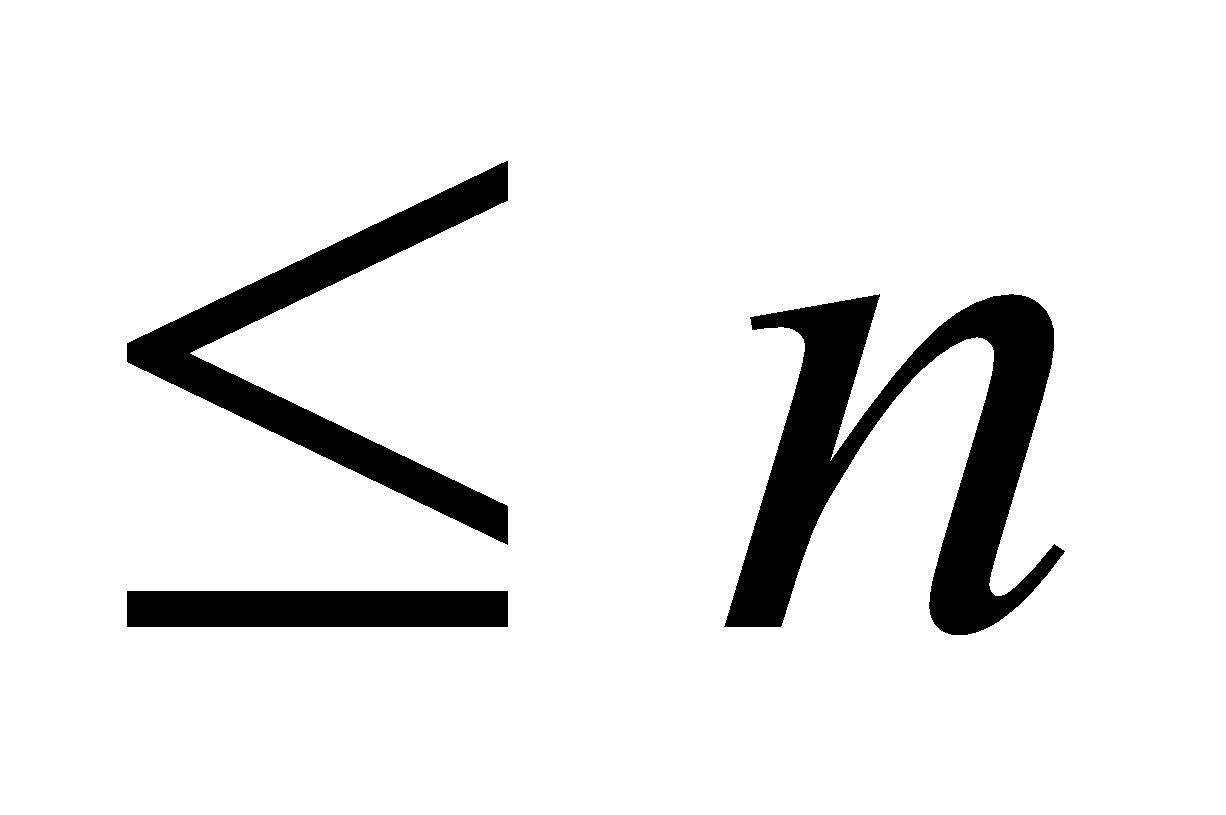, then find the value of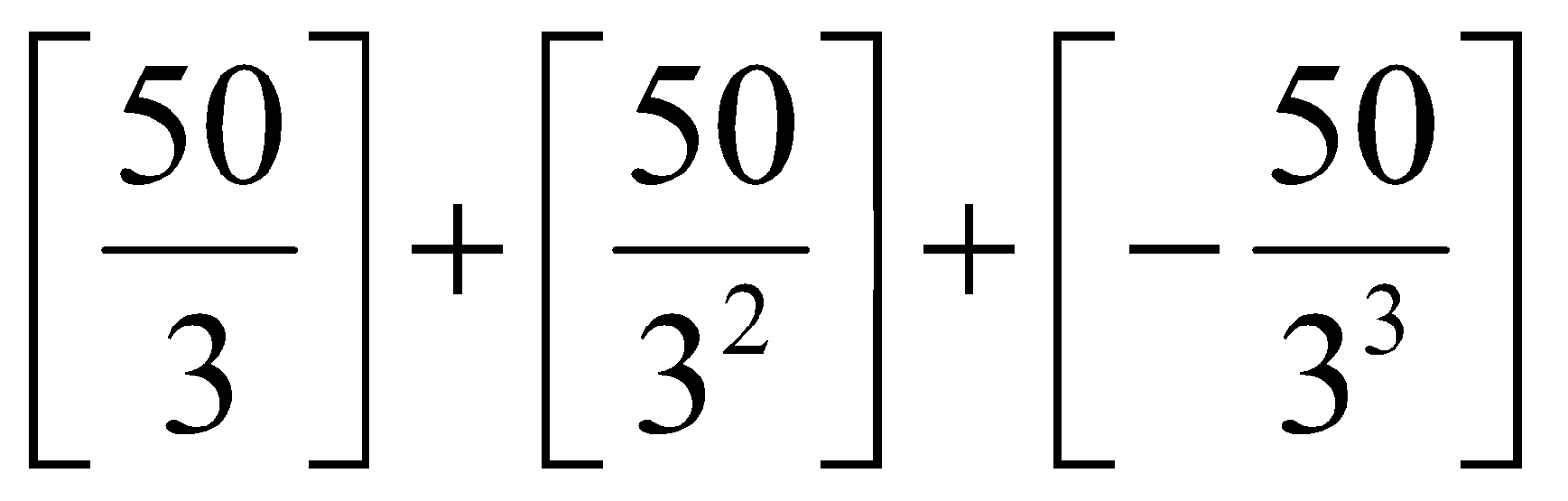1. State Fermat’s little theorem.
2. What is the value of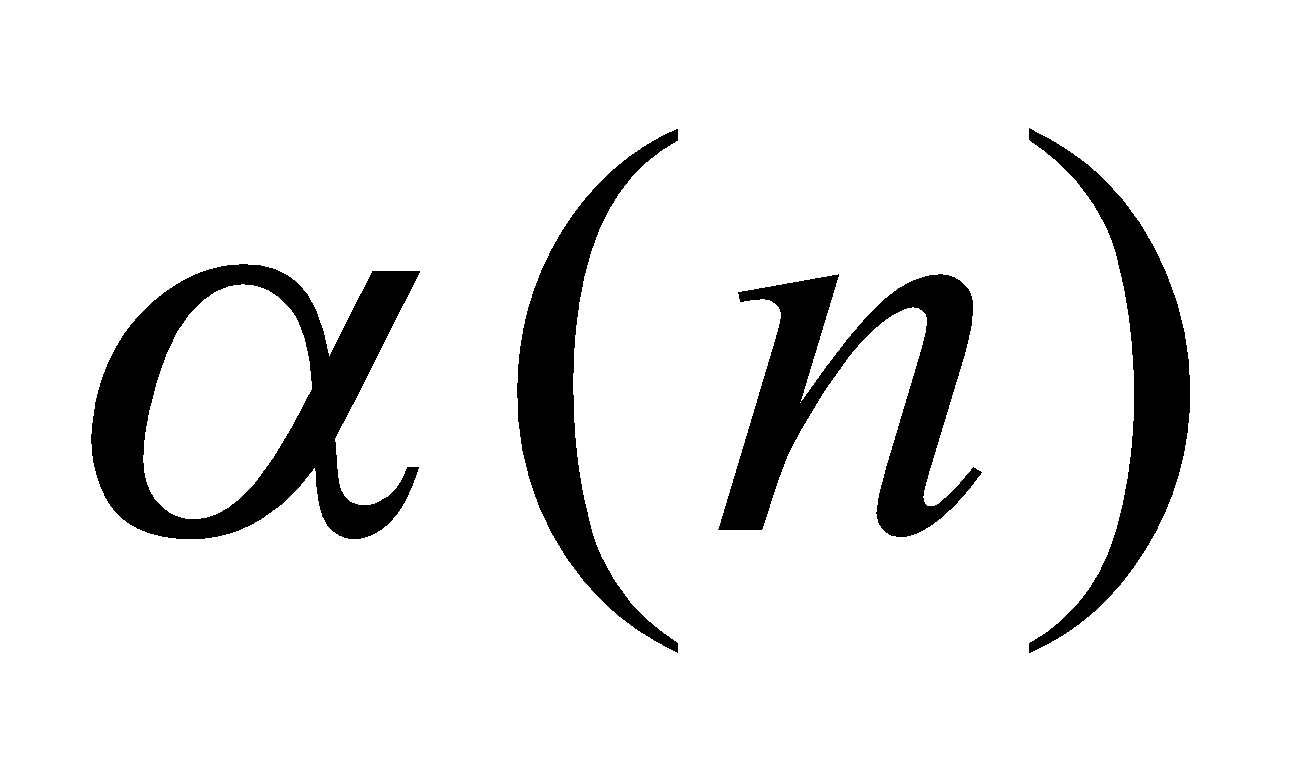, if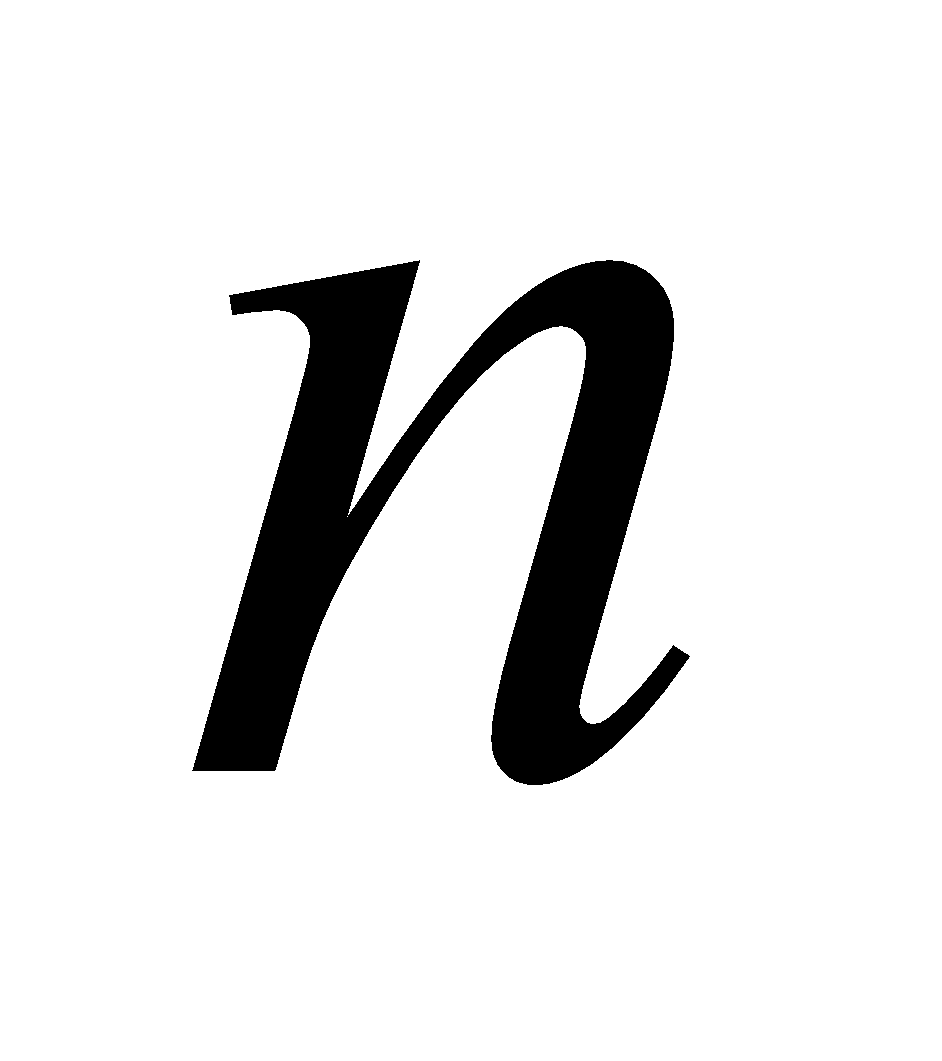is prime?
5. Answer the following questions: 2x3=6
1. Prove that every non-empty subset of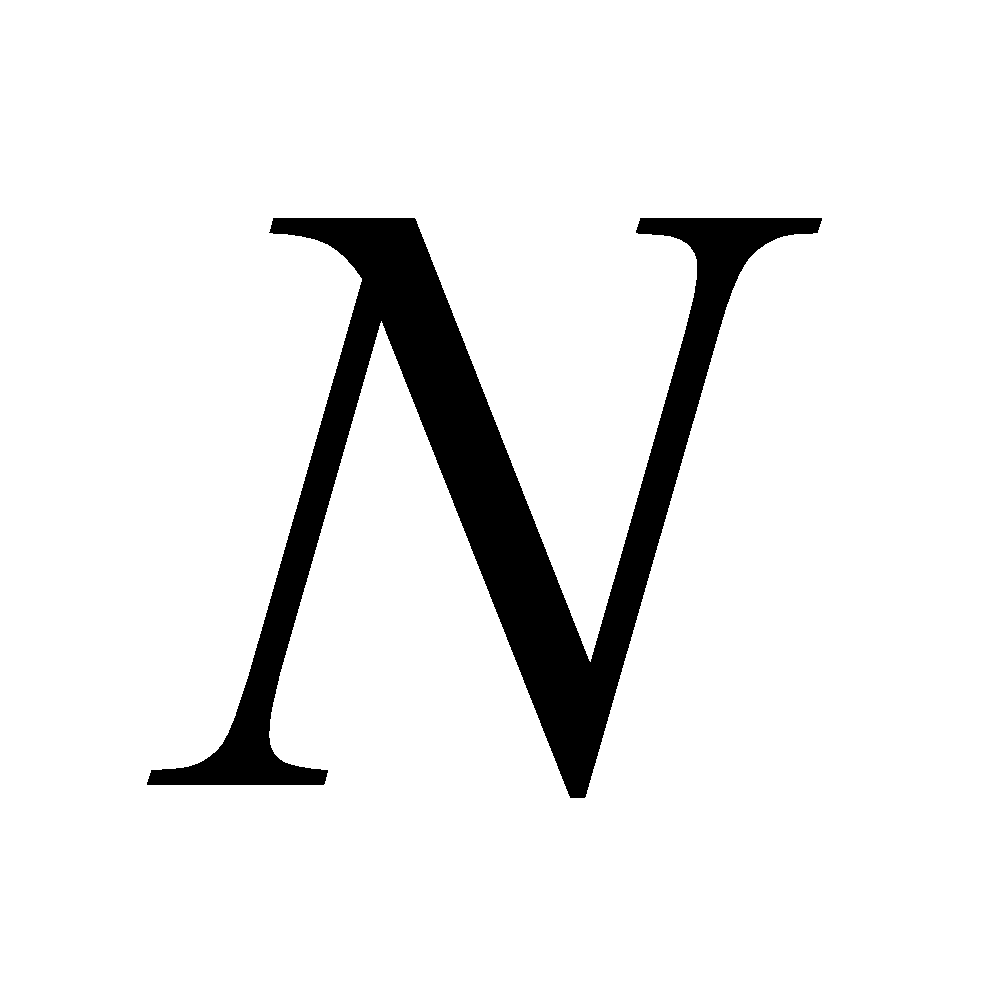contains a least element.
2. If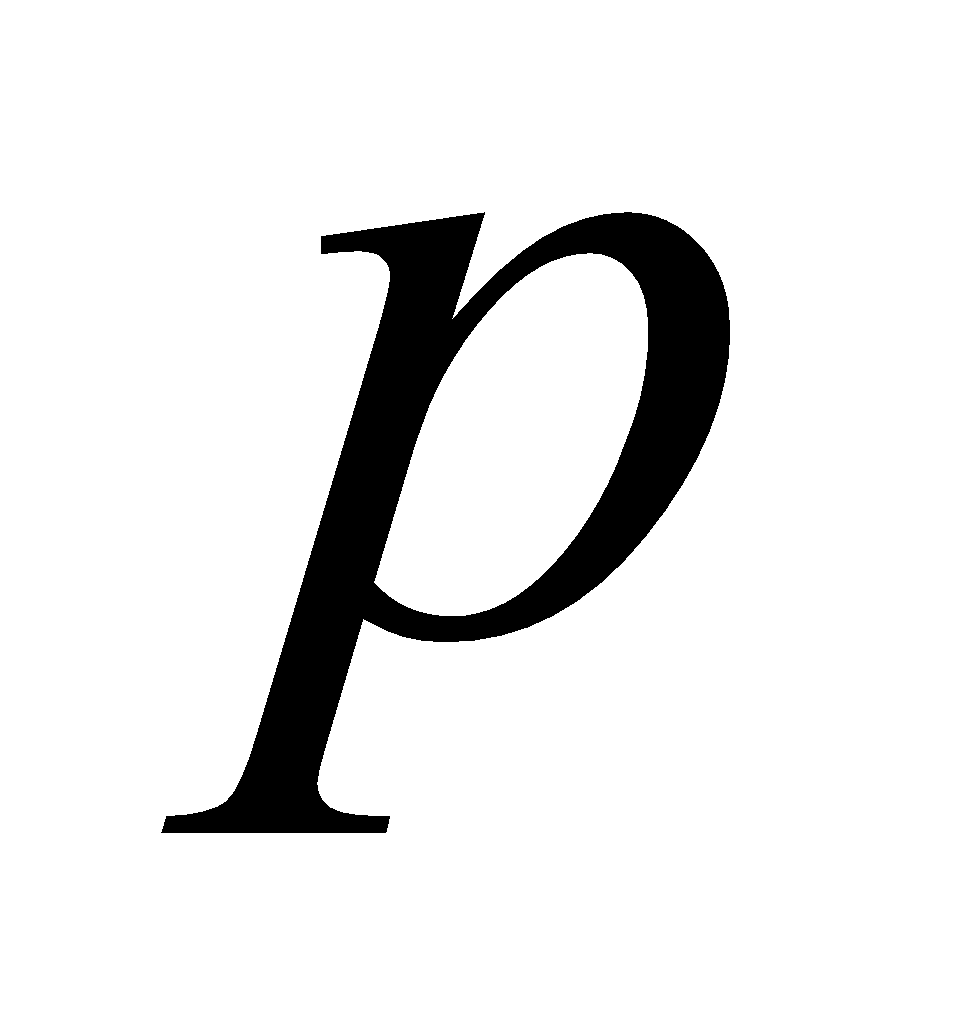is a prime and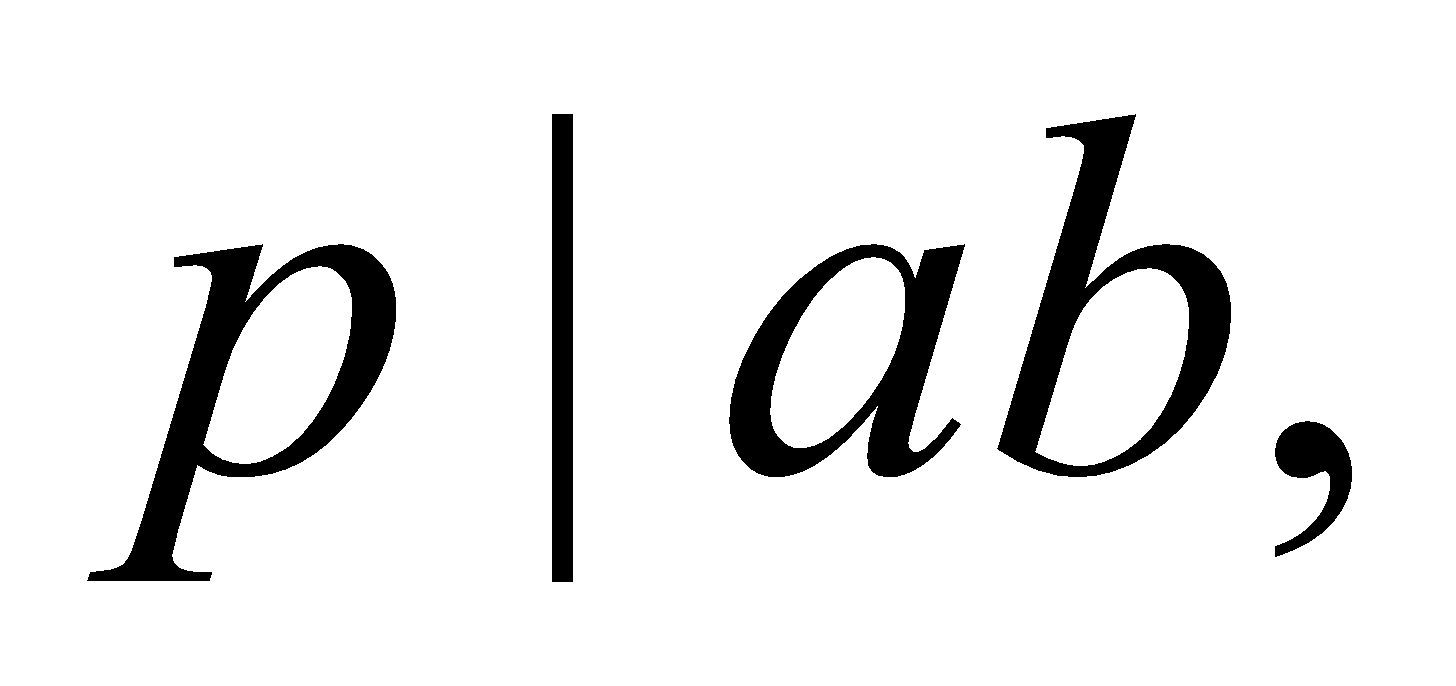then prove that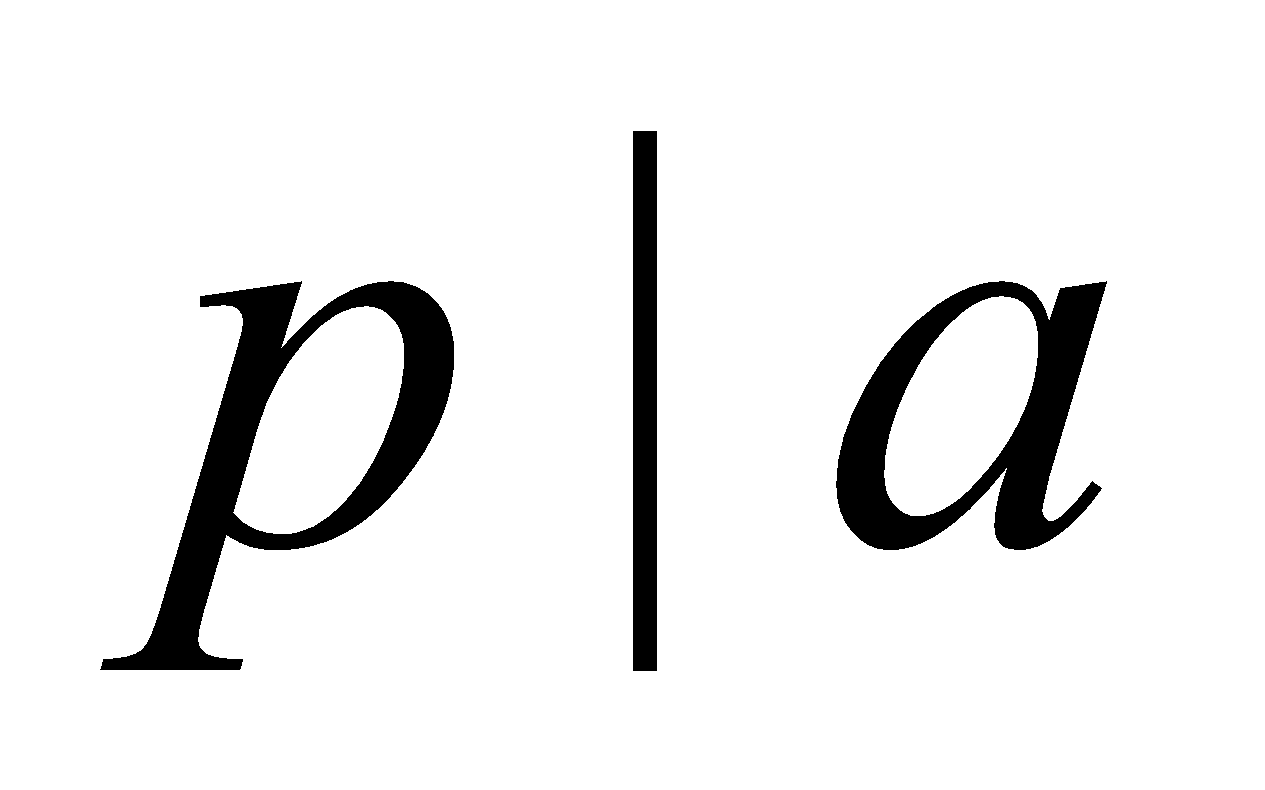or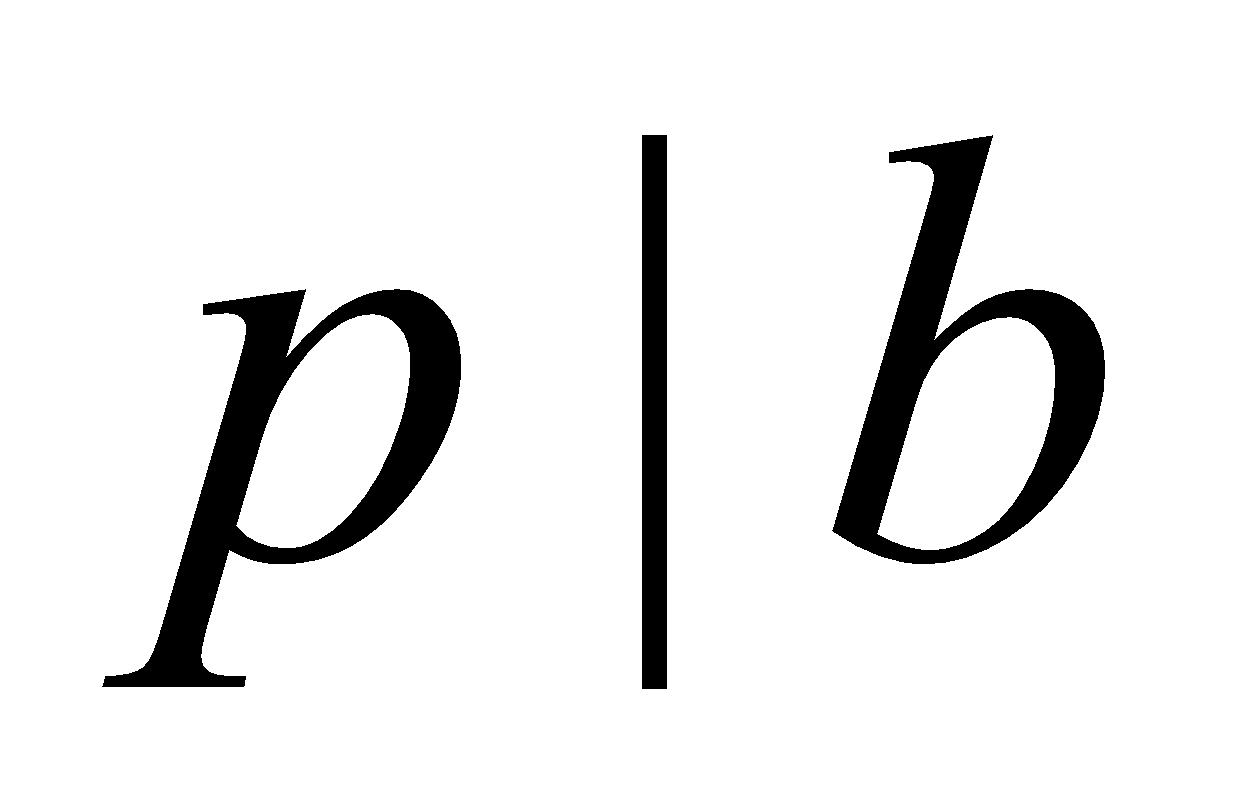, where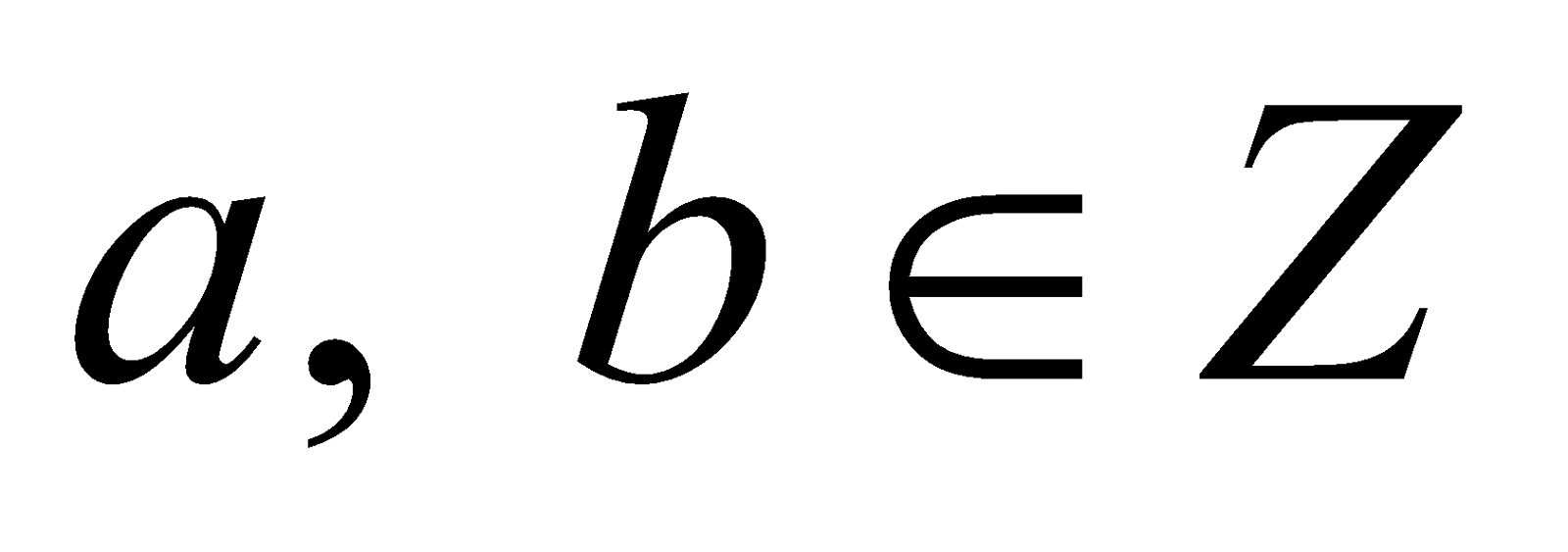.
3. For any prime, who that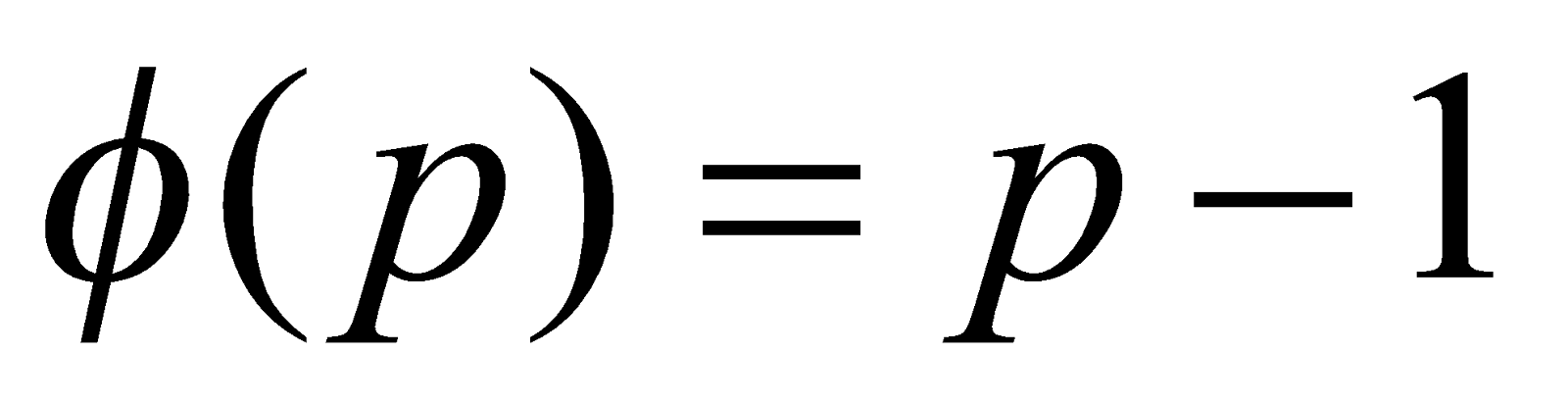.
6. Answer the following questions: 3x6=18
1. Prove that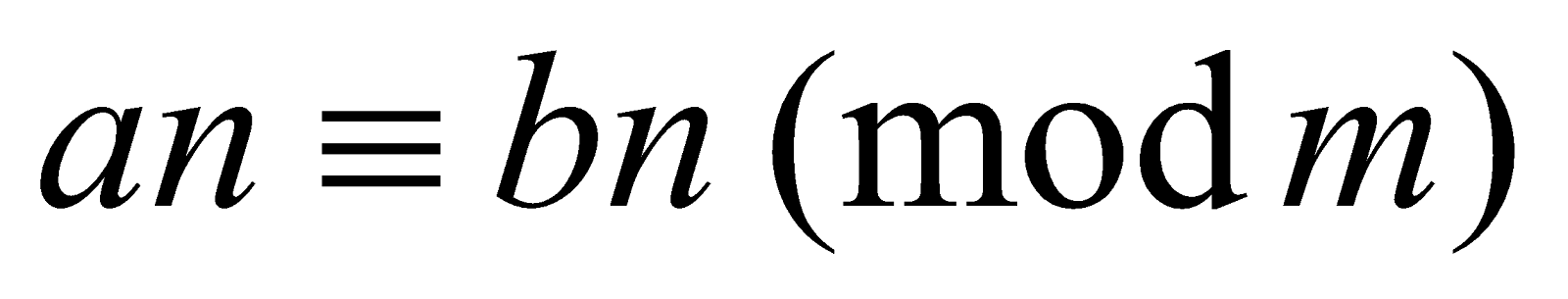, if and only if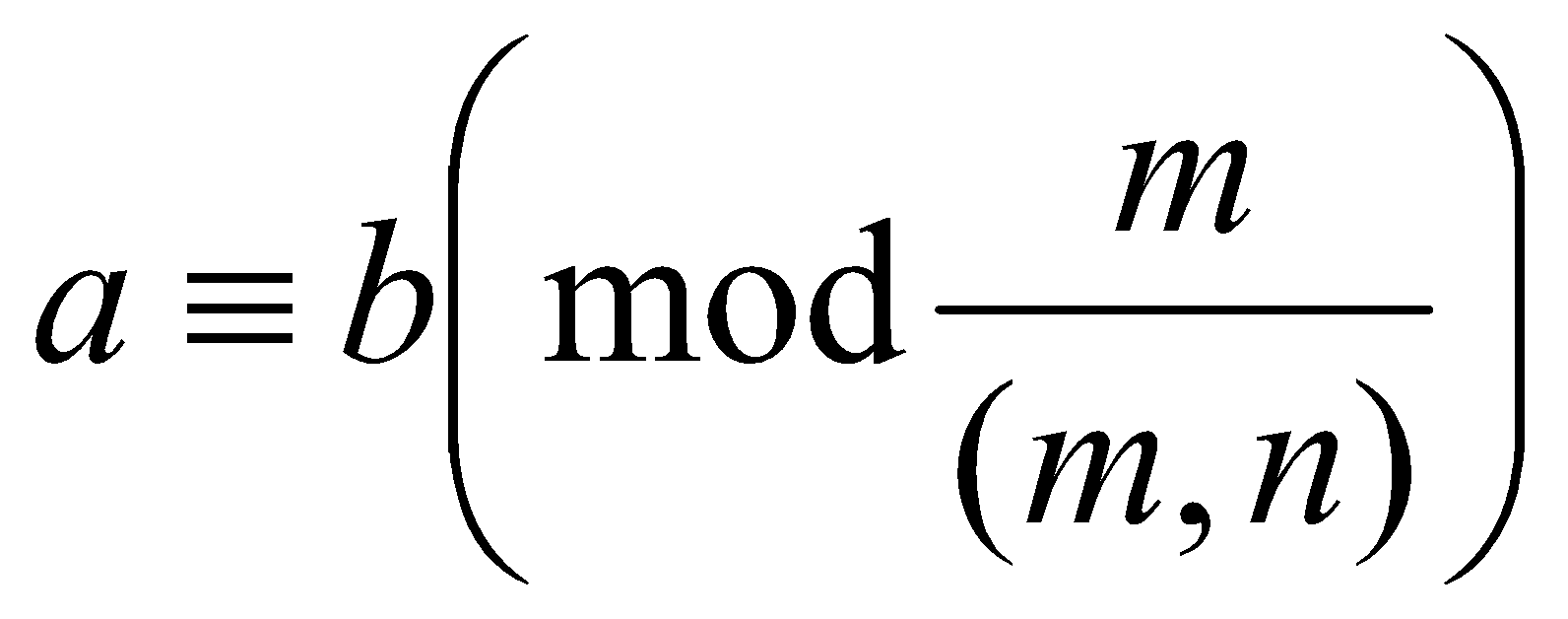1. Find the remainder when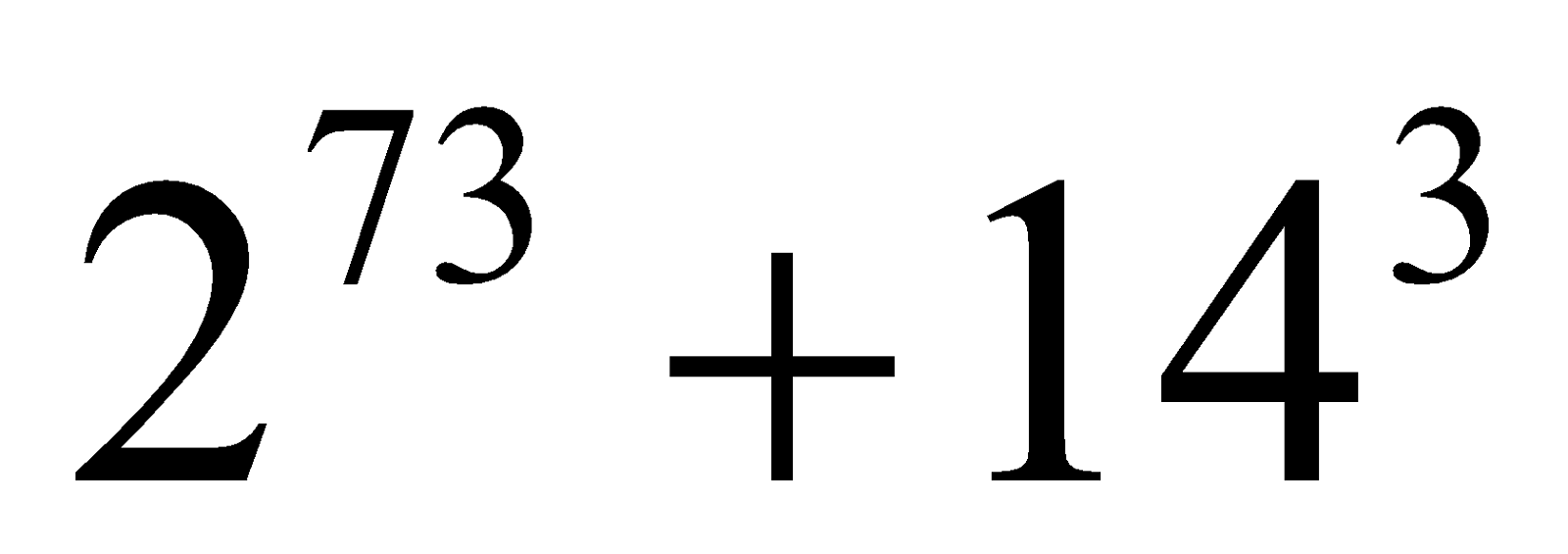is divided by 11.
2. Solve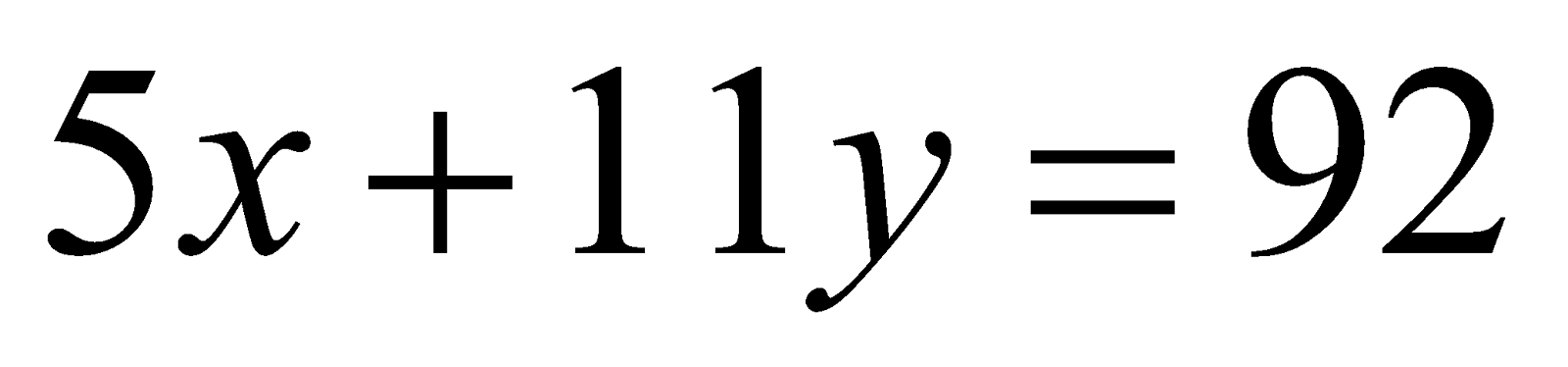.
3. Solve the system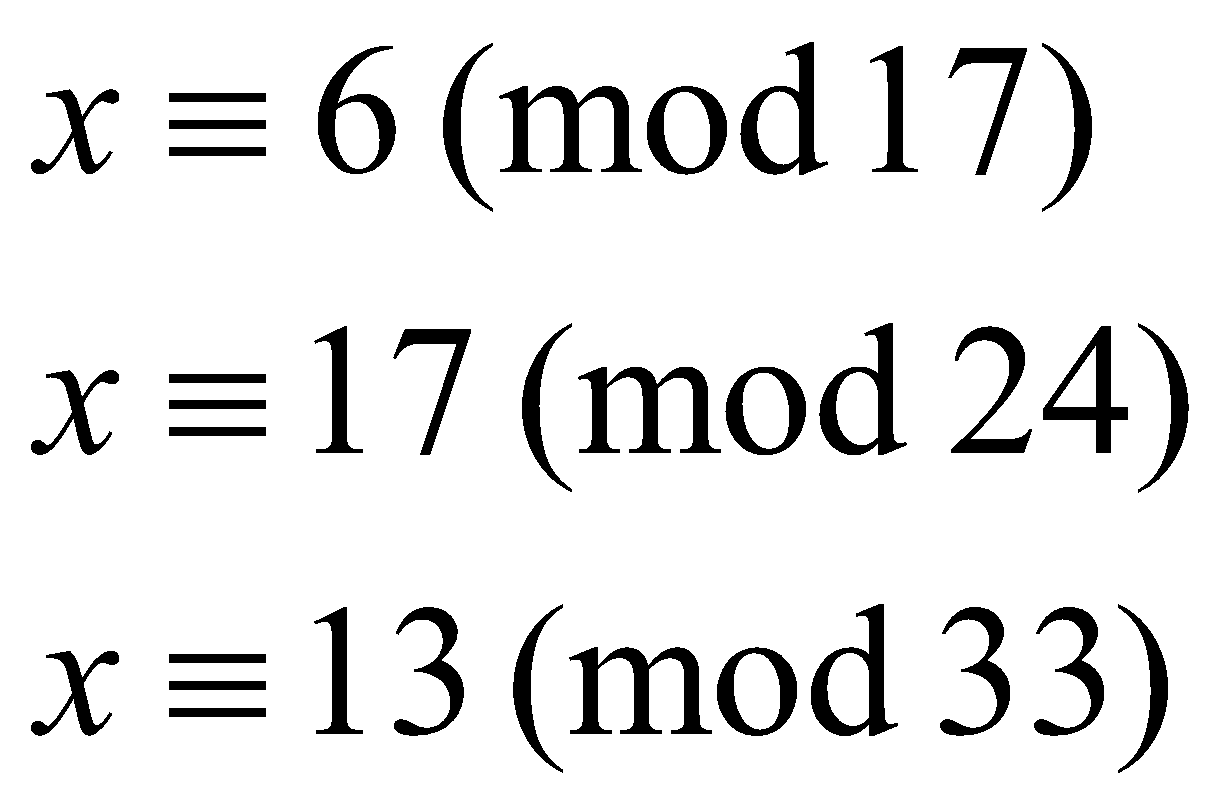1. Prove that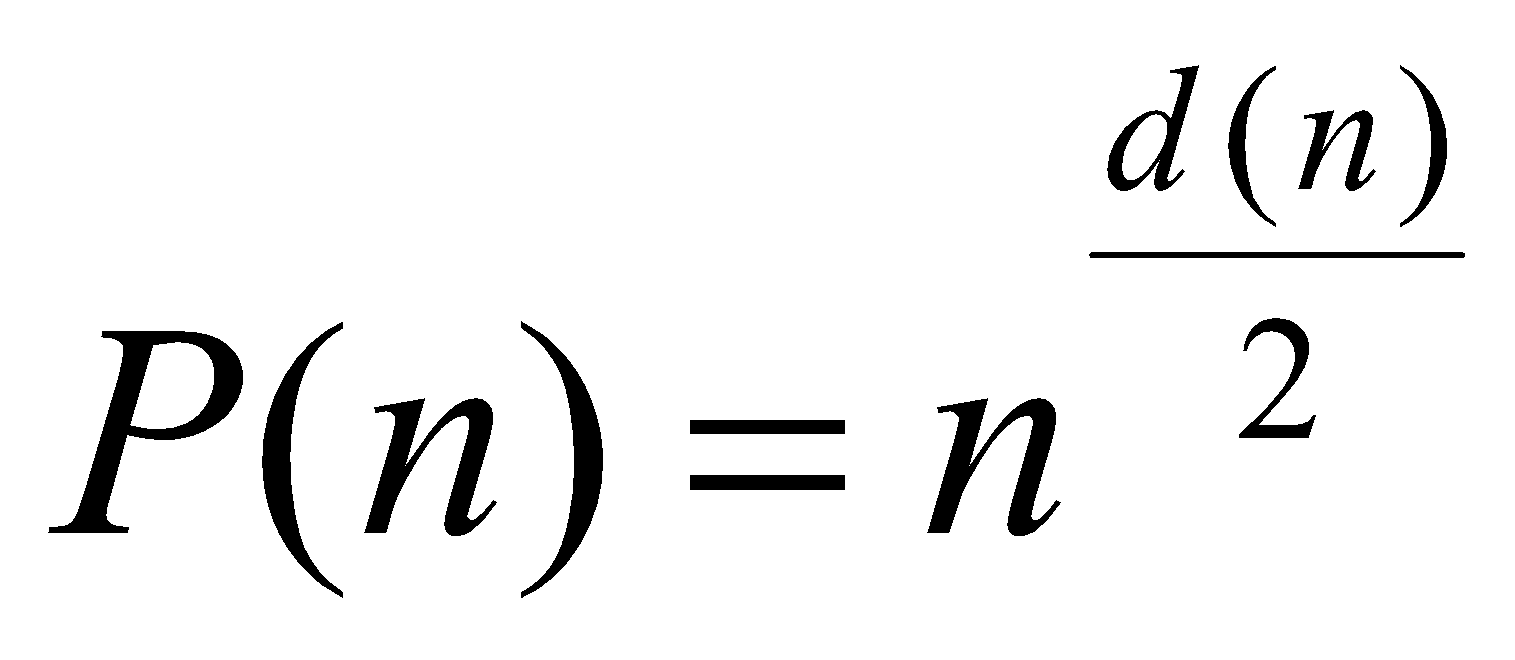.
2. Prove that there are infinitely many primes of the form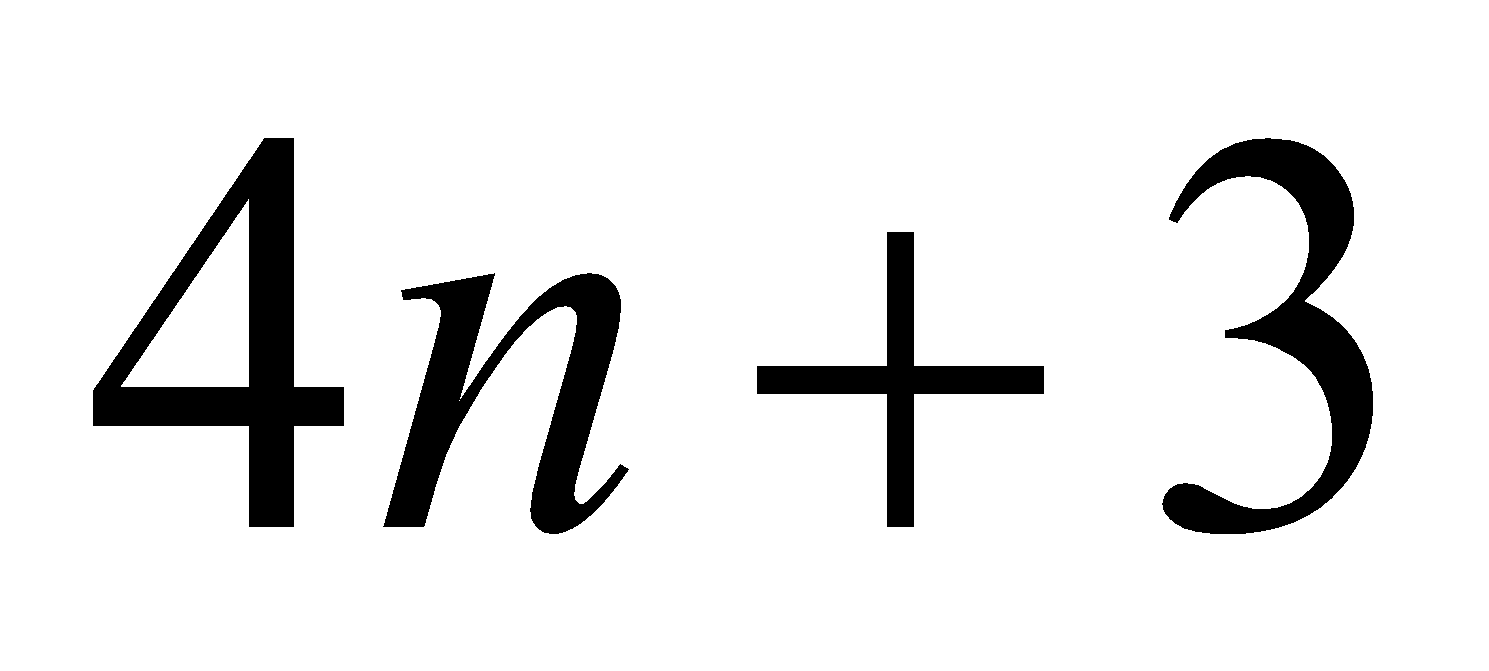.
7. Answer any three of the following: 4x3=12
1. State and prove the division algorithm.
2. Prove that every positive integer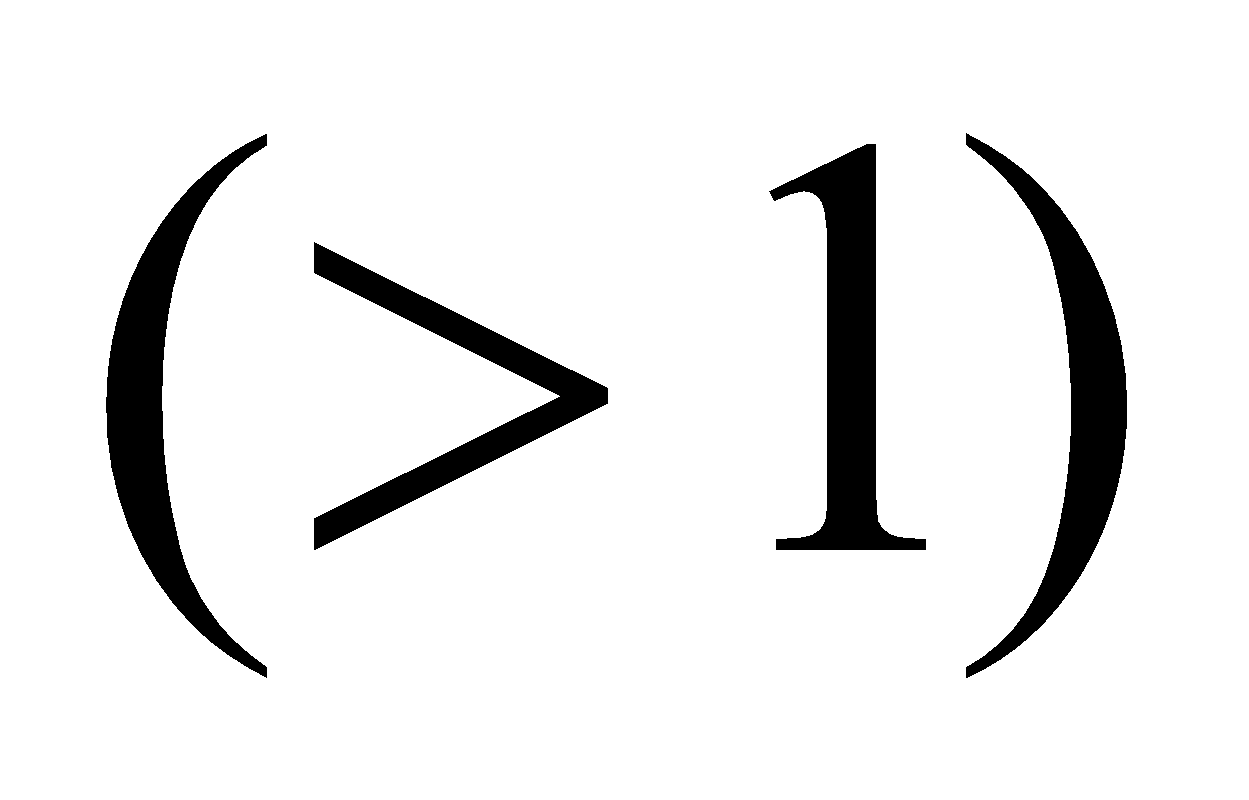can be expressed as a product of primes is unique apart from the order of factors.
3. Prove that1. State and prove Chinese remainder theorem.

***